# 6 Two Factor Classification with a Single Continuous Feature

In this Chapter we will go through some fundamentals of Exploratory Data Analysis (EDA) process of with two single continuous example features: Age and rand_Age. In Section 6.0.1 we define a new feature rand_Age as by randomly sampling from the Age distribution.

We will review the main assumptions of:

• t-test - Section 6.1
• ks-test - Section 6.3
• ANOVA Review - Section 6.6

And discuss how these statistical analyses can be used to detect statistically significant differences between groups and the role they play in Two Factor Classification between our two primary groups: patient’s with and without diabetes. We will also showcase some supportive graphics to accompany each of these statistical analysis.

Our EDA will show that while rand_Age and Age originate from the same distribution, that distribution is not the same across members with diabetes.

In section Section 6.7 We will review the Logistic Regression classification model. Along with associated correspondence between coefficients of the logistic regression and the the so called Odds Ratio. We will also review the Wald test in Section 6.7.2.

In Section 6.8 we will use R to train a logistic regression to predicting Diabetes one with feature Age. We will perform an in-depth review of the glm logistic regression model outputs in Section 6.8.1 and showcase how to utilize a model object to predict on unseen data in Section (probability-scoring-test-data-with-logit-age-model).

As a first example of a Model Evaluation Metric the Receiver Operating Characteristic (ROC) Curve in Section 6.10 is created by plotting the true positive rate (TPR) against the false positive rate (FPR) at various threshold settings.

In Section 6.11 we use the model object and the training data to obtain a threshold estimate and define predicted classes. This will lead us to review the Confusion Matrix in Section 6.12 and a variety of Model Evaluation Metrics including: Accuracy, Precision, Recall, Sensitivity, Specificity, Positive Predictive Value (PPV), Negative Predicted Value (NPV), f1-statistic, and others we will showcase in Section 6.13.

We will demonstrate that the difference between features distributions is what enables predictive models like logistic regression to effectively perform in two factor classification prediction tasks. While the p-value from the Wald statistic, is often used as an indication of feature importance within a logistic regression setting; the principals we will discuss over these next few Chapters of the EDA process will often be highly effective in determining feature selection for use in a variety of more sophisticated predictive modeling tasks going forward.

We will train a second logistic regression model with the rand_Age feature in Section 6.14.

Lastly, will again utilize the Confusion Matrix and a variety of Model Evaluation Metrics to measure model performance and develop an intuition by comparing and contrasting:

• ROC Curves - Section 6.16
• Precision-Recall Curves - Section 6.17
• Gain Curves - Section 6.18
• Lift Curves - Section 6.19
• Model Evaluation Metrics Graphs - Section 6.20

between our two logistic regression model examples.

Our goal primary goal with this chapter will be to compare and contrast these the logistic regression models and connect them back to our EDA analysis of the features to develop a functional working framework of the process that spans EDA and has impact on feature creation and selection selection, following this all the way down the line to feature impact (or lack thereof) on model performance.

$$~$$

$$~$$

First, we will load our dataset from section 3.15:

A_DATA <- readRDS('C:/Users/jkyle/Documents/GitHub/Jeff_Data_Wrangling/Week_2/DATA/A_DATA.RDS')

library('tidyverse')
#> -- Attaching packages --------------------------------------- tidyverse 1.3.1 --
#> v ggplot2 3.3.3     v purrr   0.3.4
#> v tibble  3.1.2     v dplyr   1.0.6
#> v tidyr   1.1.3     v stringr 1.4.0
#> v readr   1.4.0     v forcats 0.5.1
#> -- Conflicts ------------------------------------------ tidyverse_conflicts() --

A_DATA %>%
glimpse()
#> Rows: 101,316
#> Columns: 6
#> $SEQN <dbl> 1, 2, 3, 4, 5, 6, 7, 8, 9, 10, 11, 12, 13, 14, 15, 16,~ #>$ yr_range        <chr> "1999-2000", "1999-2000", "1999-2000", "1999-2000", "1~
#> $Age <dbl> 2, 77, 10, 1, 49, 19, 59, 13, 11, 43, 15, 37, 70, 81, ~ #>$ Gender          <chr> "Female", "Male", "Female", "Male", "Male", "Female", ~
#> $DIABETES <dbl> 0, 0, 0, 0, 0, 0, 0, 0, 0, 0, 0, 0, 1, 0, 0, 0, 0, 0, ~ #>$ AGE_AT_DIAG_DM2 <dbl> NA, NA, NA, NA, NA, NA, NA, NA, NA, NA, NA, NA, 67, NA~

As a comparison, we are going to add a feature rand_Age to the data-set. rand_Age will be sampled from the Age column in the A_DATA dataframe with replacement.

set.seed(8576309)

A_DATA$rand_Age <- sample(A_DATA$Age,
size = nrow(A_DATA),
replace = TRUE)

A_DATA %>%
glimpse()
#> Rows: 101,316
#> Columns: 7
#> $SEQN <dbl> 1, 2, 3, 4, 5, 6, 7, 8, 9, 10, 11, 12, 13, 14, 15, 16,~ #>$ yr_range        <chr> "1999-2000", "1999-2000", "1999-2000", "1999-2000", "1~
#> $Age <dbl> 2, 77, 10, 1, 49, 19, 59, 13, 11, 43, 15, 37, 70, 81, ~ #>$ Gender          <chr> "Female", "Male", "Female", "Male", "Male", "Female", ~
#> $DIABETES <dbl> 0, 0, 0, 0, 0, 0, 0, 0, 0, 0, 0, 0, 1, 0, 0, 0, 0, 0, ~ #>$ AGE_AT_DIAG_DM2 <dbl> NA, NA, NA, NA, NA, NA, NA, NA, NA, NA, NA, NA, 67, NA~
#> $rand_Age <dbl> 18, 43, 57, 60, 7, 61, 8, 76, 0, 5, 57, 59, 32, 23, 45~ Above rand_Age is defined by randomly selecting values from Age; we will show that while rand_Age and Age originate from the same distribution, that distribution is not the same across members with diabetes: $$~$$ $$~$$ ### 6.0.2dplyr statistics A_DATA %>% group_by(DIABETES) %>% summarise(mean_age = mean(Age, na.rm = TRUE), sd_age = sd(Age, na.rm = TRUE), mean_rand_Age = mean(rand_Age, na.rm=TRUE), sd_rand_Age = sd(rand_Age, na.rm=TRUE)) #> # A tibble: 3 x 5 #> DIABETES mean_age sd_age mean_rand_Age sd_rand_Age #> <dbl> <dbl> <dbl> <dbl> <dbl> #> 1 0 30.0 23.6 31.3 25.0 #> 2 1 61.5 14.8 31.2 25.0 #> 3 NA 12.0 24.5 30.9 24.7 We notice that the mean_age of the diabetics appears to be twice as much as the mean_rand_Age. We can perform some statistical analyses to confirm these two means are in-fact different, in this chapter we will review: 1. The t-test may be used to test the hypothesis that two normalish sample distributions have the same mean. 2. The Kolmogorov–Smirnov or ks-test may be used to test the hypothesis that two sample distributions were drawn from the same continuous distribution. 3. Lastly, Analysis of Variance (ANOVA) also may be used to test if two or more normalish distributions have the same mean. $$~$$ $$~$$ ## 6.1 t-test The t-test is two-sample statistical test which tests the null hypothesis that two normal distributions have the same mean. $H_O: \mu_1 = \mu_2$ where $$\mu_i$$ are the distribution means. The $$t$$-statistic is defined by: $t = \frac{\mu_1 - \mu_2}{\sqrt{S^{2}_{X_1}+S^{2}_{X_2}}}$ where $$S^{2}_{X_i}$$ is the standard error, for a given sample standard deviation. Once the t value and degrees of freedom are determined, a p-value can be found using a table of values from a t-distribution. If the calculated p-value is below the threshold chosen for statistical significance (usually at the 0.05, 0.10, or 0.01 level), then the null hypothesis is rejected in favor of the alternative hypothesis, the means are different. $$~$$ Now we will use the t-test on Age between groups of DIABETES. First we can extract lists of Ages for each type of DIABETES: DM2.Age <- (A_DATA %>% filter(DIABETES == 1))$Age

Non_DM2.Age <- (A_DATA %>%
filter(DIABETES == 0))$Age Miss_DM2.Age <- (A_DATA %>% filter(is.na(DIABETES)))$Age

Let’s compare the mean ages between the Diabetic and non-Diabetic populations with the t-test:

t_test.Age.Non_Dm2.DM2 <- t.test(Non_DM2.Age, DM2.Age,
alternative = 'two.sided',
conf.level = 0.95)

Here’s a look at the output

t_test.Age.Non_Dm2.DM2
#>
#>  Welch Two Sample t-test
#>
#> data:  Non_DM2.Age and DM2.Age
#> t = -160.7, df = 9709.2, p-value < 2.2e-16
#> alternative hypothesis: true difference in means is not equal to 0
#> 95 percent confidence interval:
#>  -31.86977 -31.10167
#> sample estimates:
#> mean of x mean of y
#>  30.04079  61.52652

The output displays that the means are: 30.0407933, 61.5265168 and the p-value is 0

Let’s review the structure of the t.test output:

str(t_test.Age.Non_Dm2.DM2)
#> List of 10
#>  $statistic : Named num -161 #> ..- attr(*, "names")= chr "t" #>$ parameter  : Named num 9709
#>   ..- attr(*, "names")= chr "df"
#>  $p.value : num 0 #>$ conf.int   : num [1:2] -31.9 -31.1
#>   ..- attr(*, "conf.level")= num 0.95
#>  $estimate : Named num [1:2] 30 61.5 #> ..- attr(*, "names")= chr [1:2] "mean of x" "mean of y" #>$ null.value : Named num 0
#>   ..- attr(*, "names")= chr "difference in means"
#>  $stderr : num 0.196 #>$ alternative: chr "two.sided"
#>  $method : chr "Welch Two Sample t-test" #>$ data.name  : chr "Non_DM2.Age and DM2.Age"
#>  - attr(*, "class")= chr "htest"

We see that the default return is a list of 10 items including:

1. statistic
2. parameter
3. p-value
4. confidence interval
5. estimate
6. null.value
7. stderr
8. alternative
9. method
10. data names

$$~$$

$$~$$

## 6.2broom

We can tidy-up the results if we utilize the broom package. Recall that broom::tidy tells R to look in the broom package for the tidy function. The tidy function in the broom library converts many base R outputs to tibbles:

broom::tidy(t_test.Age.Non_Dm2.DM2) %>%
glimpse()
#> Rows: 1
#> Columns: 10
#> $estimate <dbl> -31.48572 #>$ estimate1   <dbl> 30.04079
#> $estimate2 <dbl> 61.52652 #>$ statistic   <dbl> -160.704
#> $p.value <dbl> 0 #>$ parameter   <dbl> 9709.249
#> $conf.low <dbl> -31.86977 #>$ conf.high   <dbl> -31.10167
#> $method <chr> "Welch Two Sample t-test" #>$ alternative <chr> "two.sided"

We see that broom::tidy has transformed our list output from the t-test and made it a tibble, since it has now become a tibble we can use any other dplyr function:

library('broom')

tibble_t_test.Age.Non_Dm2.DM2 <- tidy(t_test.Age.Non_Dm2.DM2) %>%
mutate(dist_1 = "Non_DM2.Age") %>%
mutate(dist_2 = "DM2.Age")

Above, we add variables to help us remember where the data came from, so the tibble has been transformed to:

tibble_t_test.Age.Non_Dm2.DM2 %>%
glimpse()
#> Rows: 1
#> Columns: 12
#> $estimate <dbl> -31.48572 #>$ estimate1   <dbl> 30.04079
#> $estimate2 <dbl> 61.52652 #>$ statistic   <dbl> -160.704
#> $p.value <dbl> 0 #>$ parameter   <dbl> 9709.249
#> $conf.low <dbl> -31.86977 #>$ conf.high   <dbl> -31.10167
#> $method <chr> "Welch Two Sample t-test" #>$ alternative <chr> "two.sided"
#> $dist_1 <chr> "Non_DM2.Age" #>$ dist_2      <chr> "DM2.Age"

Review the p-value of the t-test between two sample distributions.

Null and alternative hypotheses

1. The null-hypothesis for the t-test is that the two population means are equal.
2. The alternative hypothesis for the t-test is that the two population means are not equal.

If the p-value is

1. greater than .05 then we accept the null-hypothesis - we are 95% certain that the two samples have the same mean.
2. less than .05 then we reject the null-hypothesis - we are not 95% certain that the two samples have the same mean.

Since the p-value is t_test.Age.Non_Dm2.DM2$p.value = 0 < .05 we reject the null-hypothesis of the t-test. The sample mean ages of the Diabetic population is statistically significantly different from the sample mean ages of the non-Diabetic population. A box pot or density plot can go along well with a t-test analysis: A_DATA %>% filter(!is.na(DIABETES)) %>% ggplot(aes(x = DIABETES, y=Age, fill=as.factor(DIABETES))) + geom_boxplot() + coord_flip() + labs(title = "Box Plot of Age by Diabetic Status", caption = paste0("t-test p-value = ", round(tibble_t_test.Age.Non_Dm2.DM2$p.value,4) ))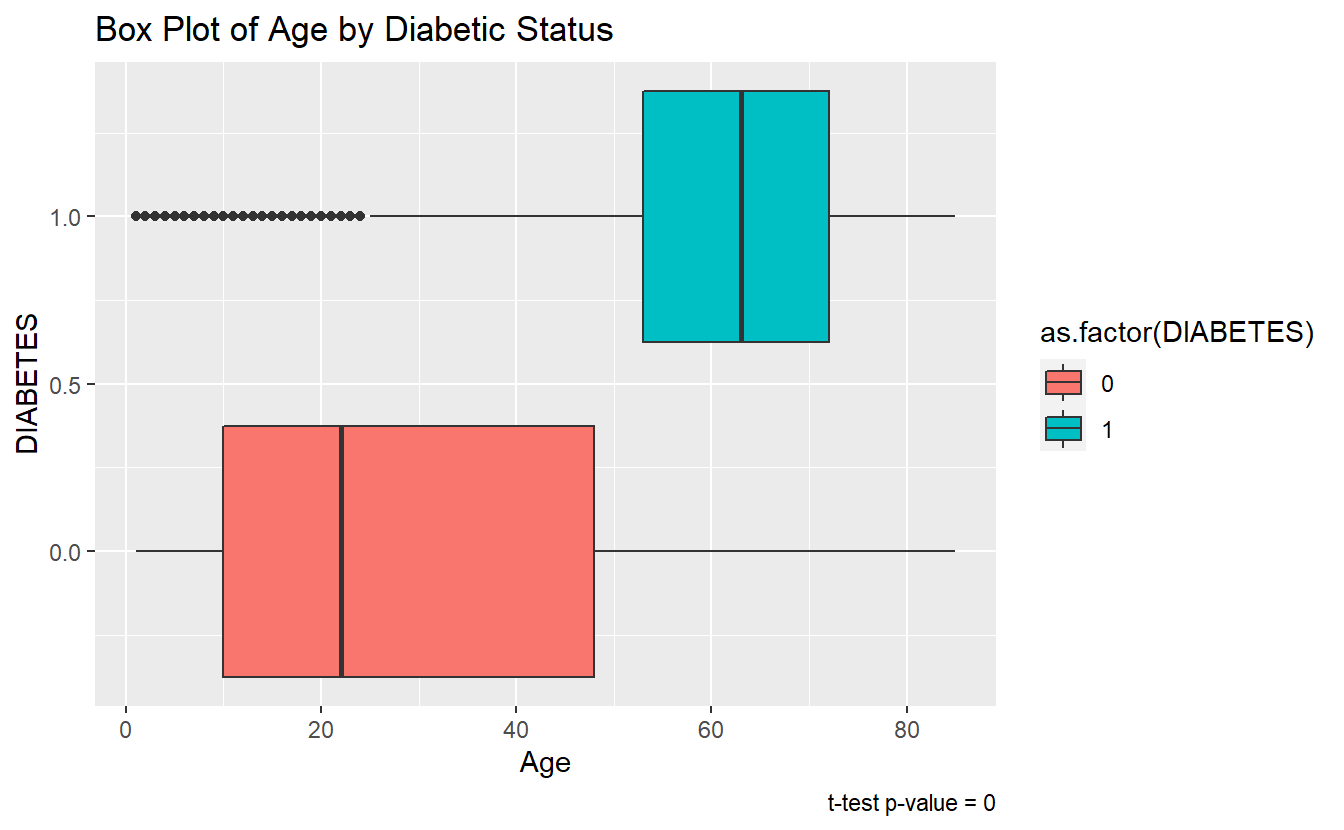$$~$$

$$~$$

## 6.3 ks-test

The two-sample Kolmogorov-Smirnov Test or ks-test will test the null-hypothesis that the two samples were drawn from the same continuous distribution.

The alternative hypothesis is that the samples were not drawn from the same continuous distribution. The ks-test can be called in a similar fashion to the t.test in section 6.1. Additionally, while the default output type of ks.test is a list again the tidy function in the broom library can be used to convert to a tibble.

tibble_ks.test.Age.Non_DM2.DM2 <- ks.test(Non_DM2.Age, DM2.Age, alternative = 'two.sided') %>%
broom::tidy()
#> Warning in ks.test(Non_DM2.Age, DM2.Age, alternative = "two.sided"): p-value
#> will be approximate in the presence of ties

tibble_ks.test.Age.Non_DM2.DM2
#> # A tibble: 1 x 4
#>   statistic p.value method                             alternative
#>       <dbl>   <dbl> <chr>                              <chr>
#> 1     0.599       0 Two-sample Kolmogorov-Smirnov test two-sided

Since the p.value is less than .05 we accept the alternative hypothesis, that the Age of Members with Diabetes was not drawn from the same distribution as Age of Members with-out Diabetes.

We can see the difference between the distributions, most clearly by the density plot:

A_DATA %>%
filter(!is.na(DIABETES))%>%
mutate(DIABETES_factor = as.factor(DIABETES)) %>%
ggplot(aes(x=Age, fill=DIABETES_factor)) +
geom_density() +
labs(title = "Density Plot - non-missing Diabetic status by Age",
caption = paste0("ks-test p-value :" , round(tibble_ks.test.Age.Non_DM2.DM2$p.value,4)))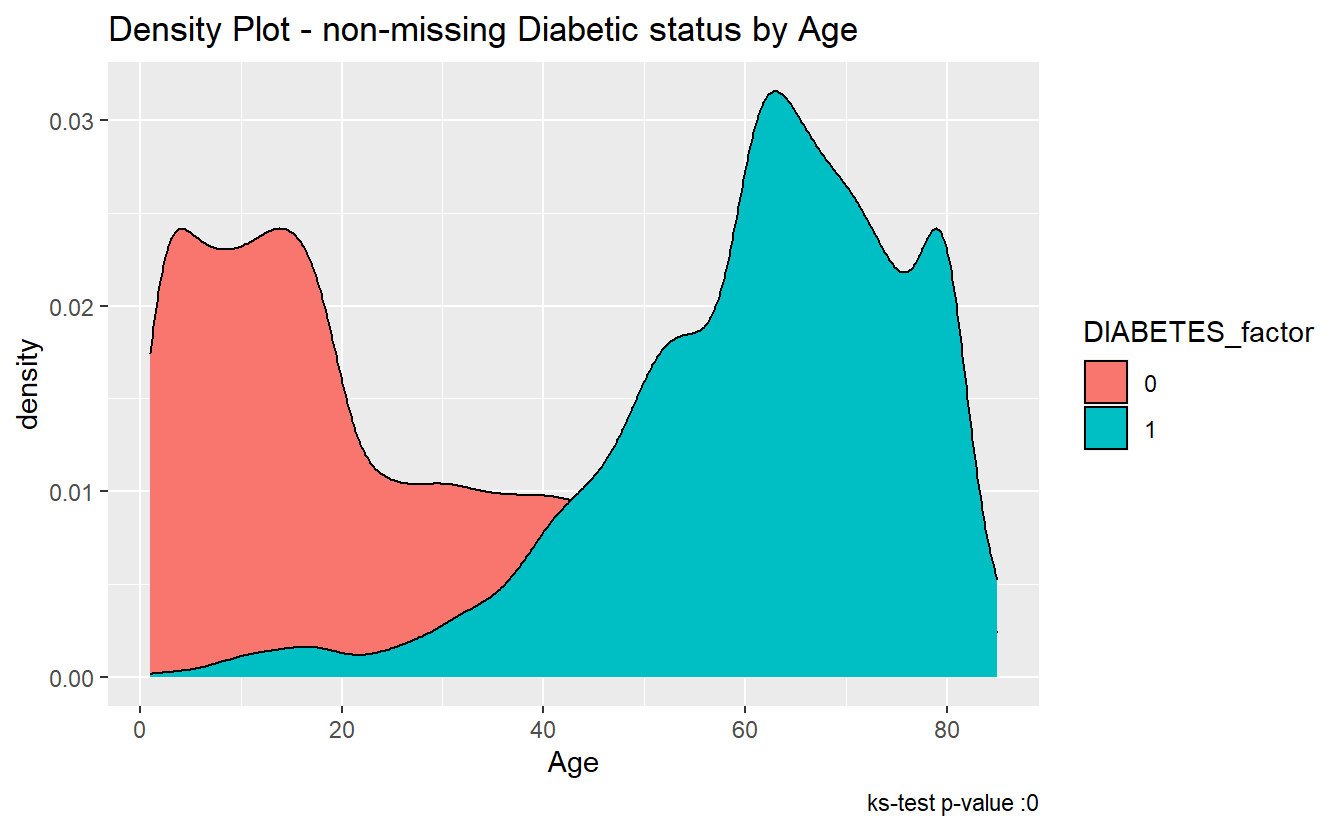The ks-statistic quantifies a distance between the empirical distribution functions (ECDF) of the two samples. And this is the plot of the two ECDFs: A_DATA %>% filter(!is.na(DIABETES))%>% mutate(DIABETES_factor = as.factor(DIABETES)) %>% ggplot(aes(x=Age, color=DIABETES_factor)) + stat_ecdf() + labs(title = "K-S Test: Age - Diabetics Vs non-Diabetics", caption = paste0("ks-test p-value :" , round(tibble_ks.test.Age.Non_DM2.DM2$p.value,4)))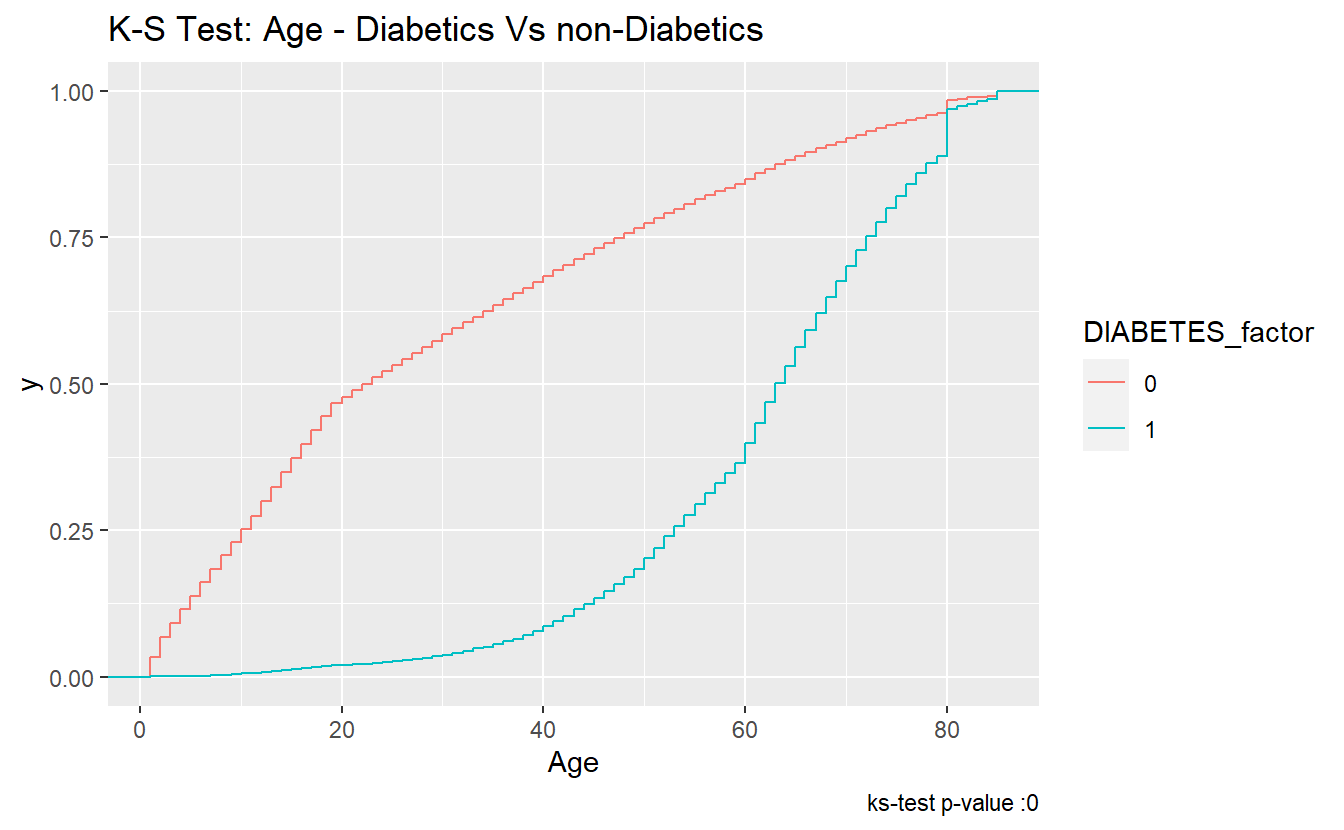$$~$$

$$~$$

## 6.4rand_Age

As we did with Age above, we can extract lists of rand_Ages for each type of DIABETES:

DM2.rand_Age <- (A_DATA %>%
filter(DIABETES == 1))$rand_Age Non_DM2.rand_Age <- (A_DATA %>% filter(DIABETES == 0))$rand_Age

Miss_DM2.rand_Age <- (A_DATA %>%
filter(is.na(DIABETES)))$rand_Age ### 6.4.1t.test Let’s compare the mean rand_Ages between the Diabetic and non-Diabetic populations: t_test.rand_Age.Non_Dm2.DM2 <- t.test(Non_DM2.rand_Age, DM2.rand_Age, alternative = 'two.sided', conf.level = 0.95) t_test.rand_Age.Non_Dm2.DM2 #> #> Welch Two Sample t-test #> #> data: Non_DM2.rand_Age and DM2.rand_Age #> t = 0.094855, df = 7878.6, p-value = 0.9244 #> alternative hypothesis: true difference in means is not equal to 0 #> 95 percent confidence interval: #> -0.5874002 0.6471383 #> sample estimates: #> mean of x mean of y #> 31.25243 31.22257 tibble_t_test.rand_Age.Non_Dm2.DM2 <- broom::tidy(t_test.rand_Age.Non_Dm2.DM2) %>% mutate(dist_1 = "Non_DM2.rand_Age") %>% mutate(dist_2 = "DM2.rand_Age") tibble_t_test.rand_Age.Non_Dm2.DM2 %>% glimpse() #> Rows: 1 #> Columns: 12 #>$ estimate    <dbl> 0.02986907
#> $estimate1 <dbl> 31.25243 #>$ estimate2   <dbl> 31.22257
#> $statistic <dbl> 0.09485536 #>$ p.value     <dbl> 0.9244321
#> $parameter <dbl> 7878.617 #>$ conf.low    <dbl> -0.5874002
#> $conf.high <dbl> 0.6471383 #>$ method      <chr> "Welch Two Sample t-test"
#> $alternative <chr> "two.sided" #>$ dist_1      <chr> "Non_DM2.rand_Age"
#> $dist_2 <chr> "DM2.rand_Age" Note that each of tibble_t_test.Age.Non_Dm2.DM2 and tibble_t_test.rand_Age.Non_Dm2.DM2 are each one row, if we want to combine these into a single dataset we can do that with the bind_rows function: bind_rows(tibble_t_test.Age.Non_Dm2.DM2, tibble_t_test.rand_Age.Non_Dm2.DM2) %>% select(dist_1, dist_2, p.value, estimate1, estimate2, conf.low, estimate, conf.high) #> # A tibble: 2 x 8 #> dist_1 dist_2 p.value estimate1 estimate2 conf.low estimate conf.high #> <chr> <chr> <dbl> <dbl> <dbl> <dbl> <dbl> <dbl> #> 1 Non_DM2.Age DM2.Age 0 30.0 61.5 -31.9 -31.5 -31.1 #> 2 Non_DM2.ran~ DM2.rand~ 0.924 31.3 31.2 -0.587 0.0299 0.647 The story here is different, since tibble_t_test.rand_Age.Non_Dm2.DM2$p.value = 0.9244321 is greater than .05 we accept the null-hypothesis and assume the sample mean of rand_Age is the same for both the Diabetic and non-Diabetic populations.

A_DATA %>%
filter(!is.na(DIABETES)) %>%
mutate(DIABETES_factor = as.factor(DIABETES)) %>%
ggplot(aes(x= rand_Age, fill=DIABETES_factor)) +
geom_boxplot() +
labs(title = "Box Plot - Diabetic status by rand_Age",
caption = paste0("t-test p-value = ", round(tibble_t_test.rand_Age.Non_Dm2.DM2$p.value,40) ))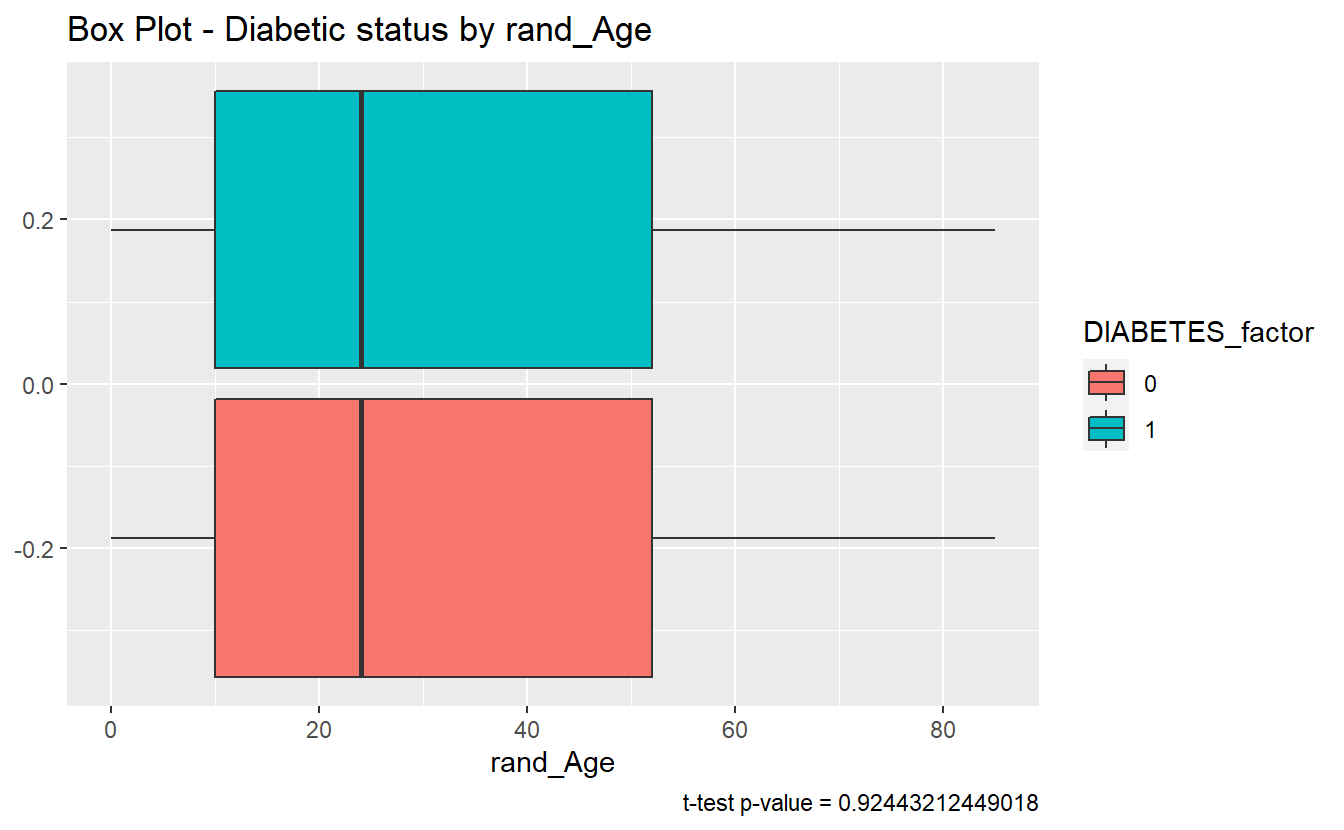### 6.4.2ks.test We can review the ks.test test for rand_Age: tibble_ks_test.rand_Age.non_DM2.DM2 <- ks.test(Non_DM2.rand_Age, DM2.rand_Age, alternative = 'two.sided') %>% broom::tidy() #> Warning in ks.test(Non_DM2.rand_Age, DM2.rand_Age, alternative = "two.sided"): #> p-value will be approximate in the presence of ties tibble_ks_test.rand_Age.non_DM2.DM2 #> # A tibble: 1 x 4 #> statistic p.value method alternative #> <dbl> <dbl> <chr> <chr> #> 1 0.00563 0.988 Two-sample Kolmogorov-Smirnov test two-sided Furthermore, since the p.value of the ks.test is 0.9881447 we accept the null-hypothesis; and assume the distributions of rand_Age of both the diabetics and non-diabetics were sampled from the same continuous distribution We can see in the density plot the distributions are not much different: A_DATA %>% filter(!is.na(DIABETES))%>% mutate(DIABETES_factor = as.factor(DIABETES)) %>% ggplot(aes(x=rand_Age, fill=DIABETES_factor)) + geom_density() + labs(title = "Density Plot - rand_Age by Diabetes", caption = paste0('ks-test p-value : ' , round(tibble_ks_test.rand_Age.non_DM2.DM2$p.value,4)))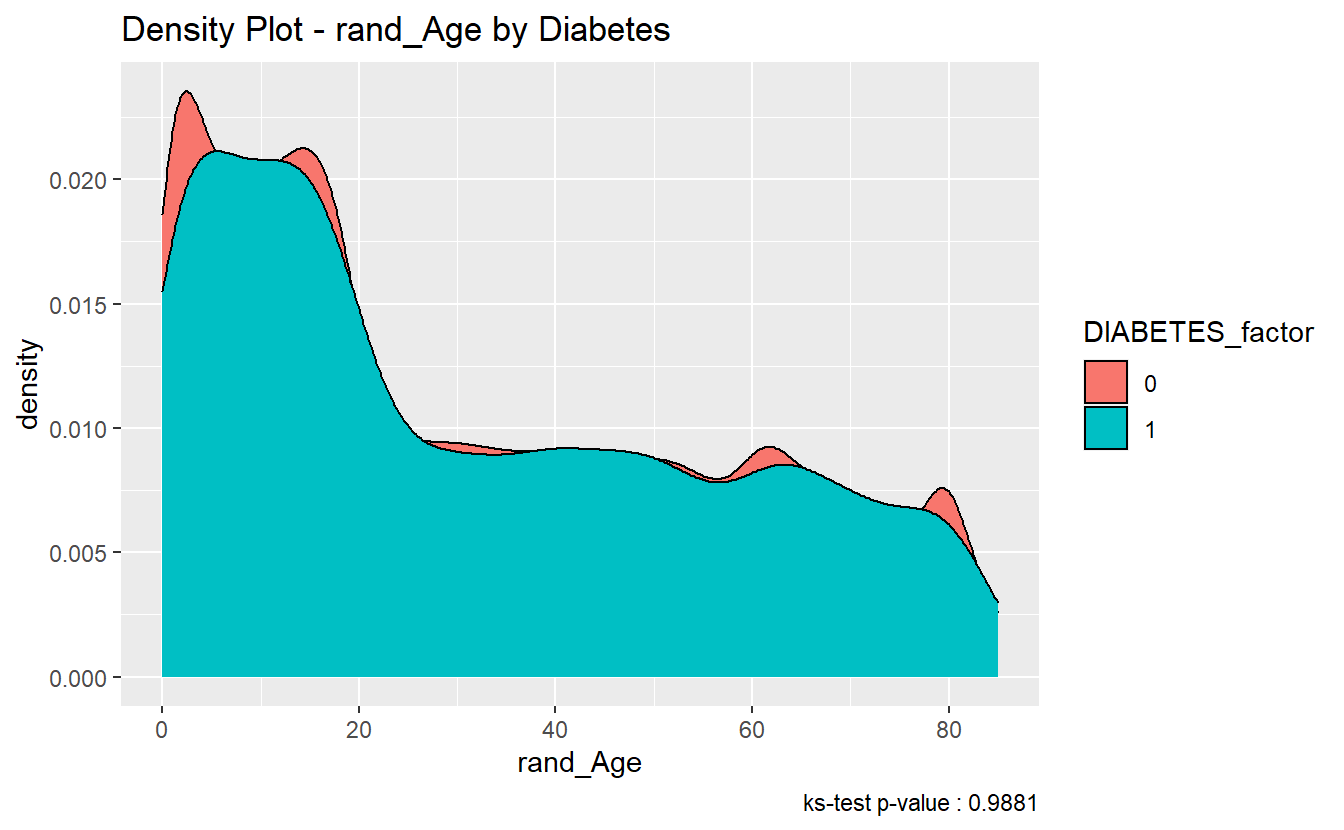And here two ECDFs are almost on top of each other:

A_DATA %>%
filter(!is.na(DIABETES))%>%
mutate(DIABETES_factor = as.factor(DIABETES)) %>%
ggplot(aes(x=rand_Age, color=DIABETES_factor)) +
stat_ecdf() +
labs(title = "K-S Test: rand_Age - Diabetics Vs non-Diabetics",
caption = paste0("ks-test p-value :" , round(tibble_ks_test.rand_Age.non_DM2.DM2$p.value,4)))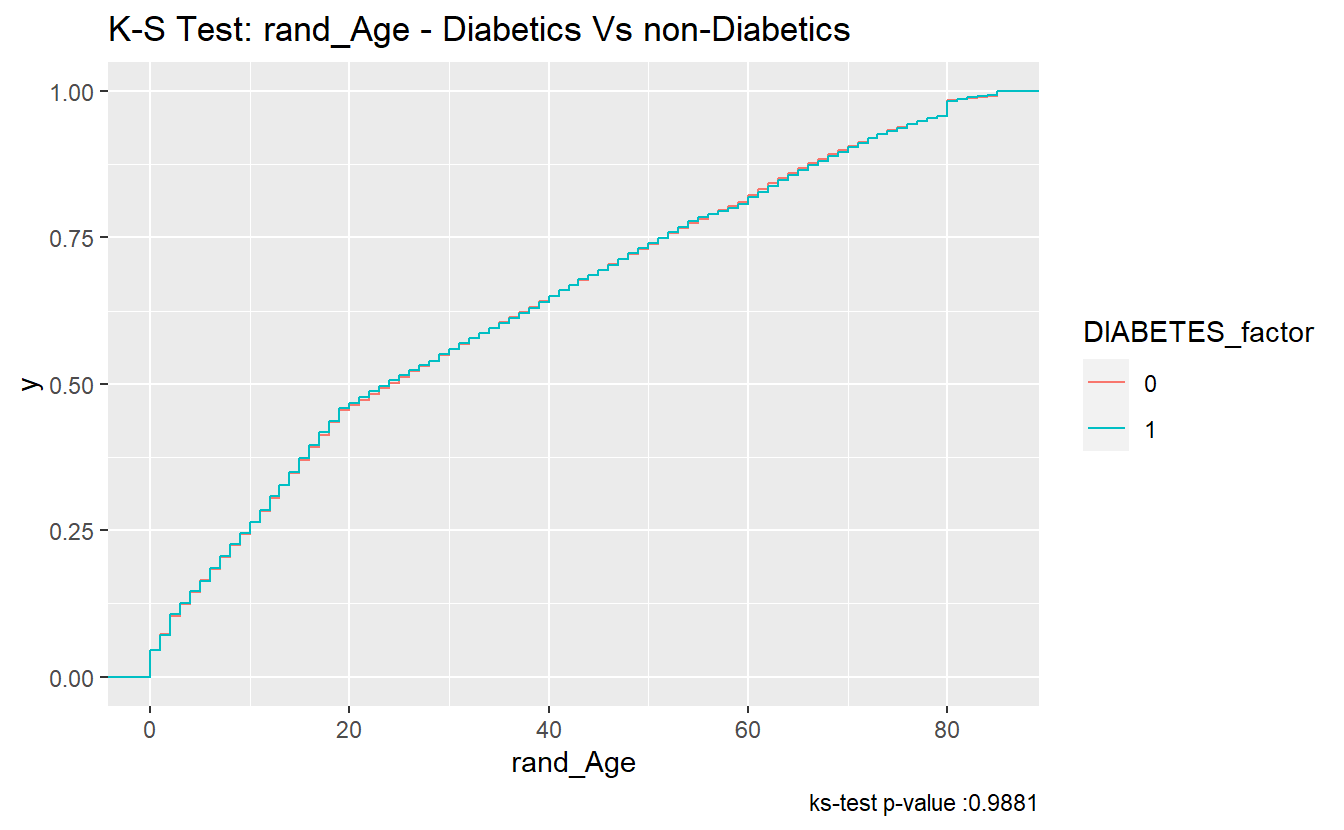### 6.4.3 Discussion Question For distributions x and y is it possible that: 1. t.test(x,y)$p.value > .05 & ks.test(x,y)$p.value < .05 ? 2. What about for t.test(x,y)$p.value < .05 & ks.test(x,y)$p.value > .05 ? $$~$$ $$~$$ #### 6.4.3.1 Compare “Missing” Diabetic class among Age and rand_Age ##### 6.4.3.1.1 Histograms Histograms can sometimes be helpful in getting started: hist_plot_1 <- A_DATA %>% mutate(DIABETES_factor = as.factor(DIABETES)) %>% ggplot(aes(x=Age, fill=DIABETES_factor)) + geom_histogram(alpha=0.6, position = 'identity' , binwidth = 5 ) + labs(title = "Histogram - Diabetic status by Age") hist_plot_1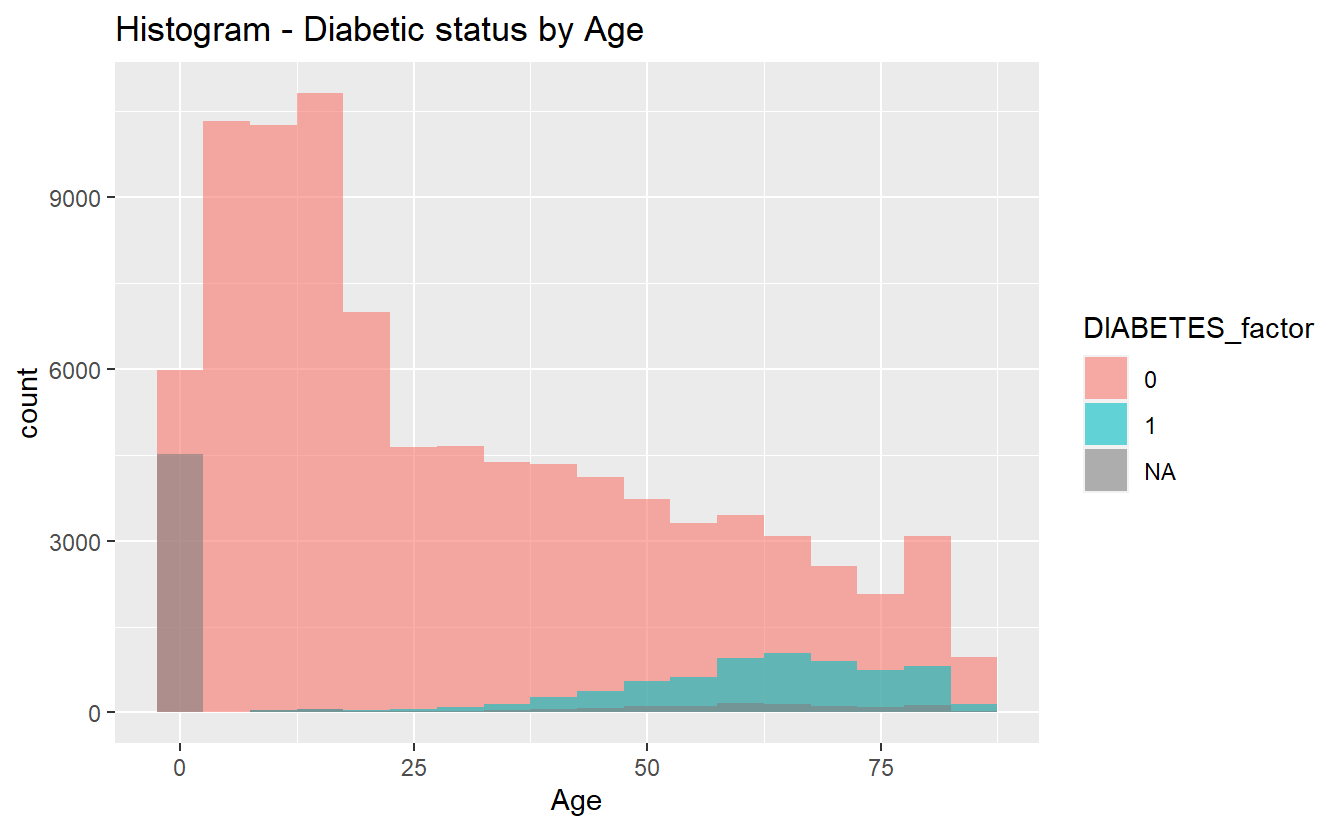plotly::ggplotly(hist_plot_1) We will once again review t-test analysis: ##### 6.4.3.1.2 t-test tibble_t_test.Age.Non_Dm2.Miss_DM2 <- broom::tidy(t.test( Miss_DM2.Age, Non_DM2.Age)) %>% mutate(dist_1 = "Miss_DM2.Age") %>% mutate(dist_2 = "Non_DM2.Age")  tibble_t_test.Age.Dm2.Miss_DM2 <- broom::tidy(t.test(DM2.Age, Miss_DM2.Age)) %>% mutate(dist_1 = "DM2.Age") %>% mutate(dist_2 = "Miss_DM2.Age") tibble_ttests <- bind_rows(tibble_t_test.Age.Non_Dm2.DM2, tibble_t_test.Age.Non_Dm2.Miss_DM2, tibble_t_test.Age.Dm2.Miss_DM2) tibble_ttests %>% select(dist_1, dist_2, p.value, estimate1, estimate2, conf.low, estimate, conf.high) #> # A tibble: 3 x 8 #> dist_1 dist_2 p.value estimate1 estimate2 conf.low estimate conf.high #> <chr> <chr> <dbl> <dbl> <dbl> <dbl> <dbl> <dbl> #> 1 Non_DM2.Age DM2.Age 0 30.0 61.5 -31.9 -31.5 -31.1 #> 2 Miss_DM2.A~ Non_DM2.A~ 0 12.0 30.0 -18.7 -18.1 -17.4 #> 3 DM2.Age Miss_DM2.~ 0 61.5 12.0 48.8 49.5 50.3 ###### 6.4.3.1.2.1 box-plots We can see the difference between each of the three Age means at once we can make the plots interactive if we utilize plotly::ggplotly, a good supporting plot to go along with the above table might be the box-plot: box_plot_1 <- A_DATA %>% mutate(DIABETES_factor = as.factor(DIABETES)) %>% ggplot(aes(x=DIABETES_factor, y=Age , fill=DIABETES_factor)) + geom_boxplot() + coord_flip() + labs(title = "Box Plot - Diabetic status by Age") box_plot_1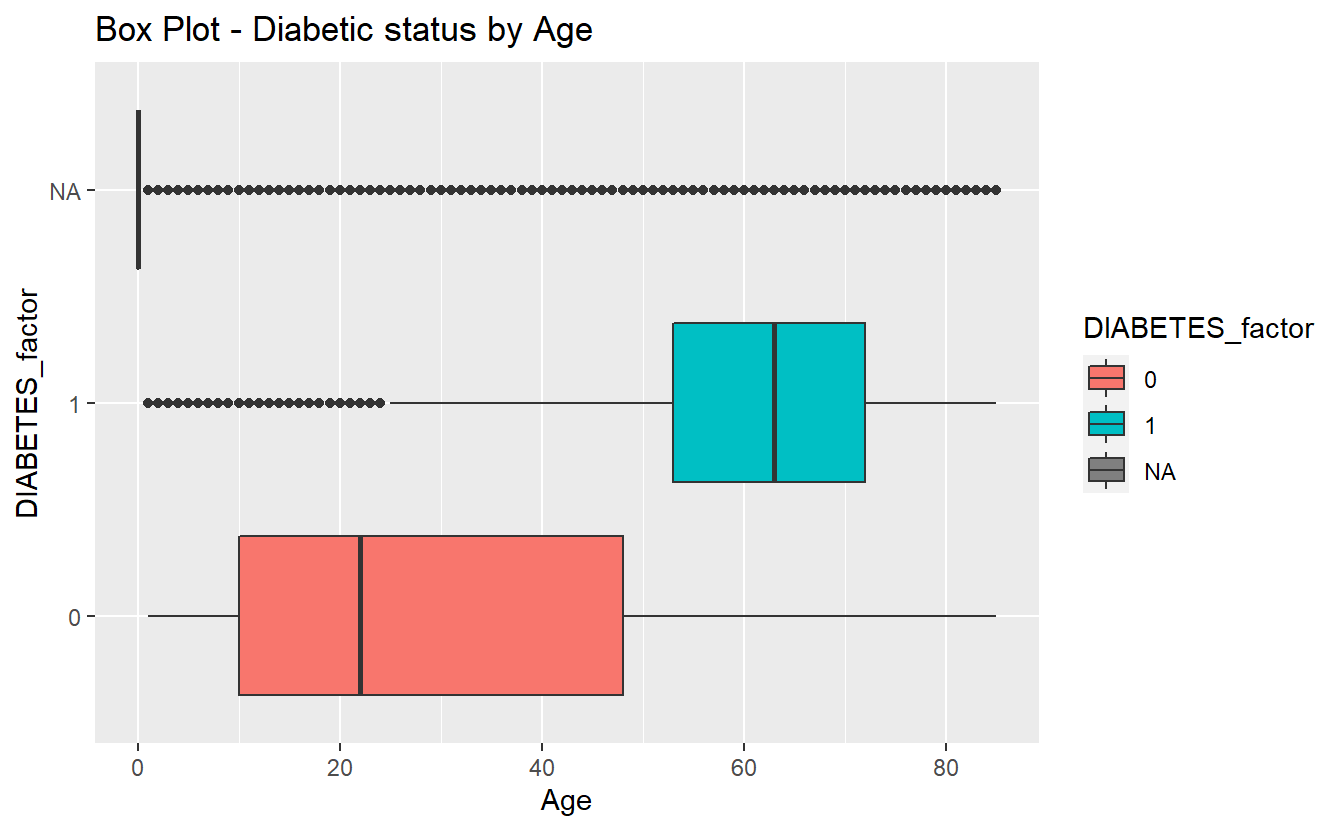plotly::ggplotly(box_plot_1) ##### 6.4.3.1.3 ks-test tibble_ks_test.Age.Non_Dm2.DM2 <- ks.test(Non_DM2.Age, DM2.Age, alternative = 'two.sided') %>% broom::tidy() %>% mutate(dist_1 = "Non_DM2.Age") %>% mutate(dist_2 = "DM2.Age") #> Warning in ks.test(Non_DM2.Age, DM2.Age, alternative = "two.sided"): p-value #> will be approximate in the presence of ties tibble_ks_test.Age.Non_Dm2.Miss_DM2 <- ks.test( Miss_DM2.Age, Non_DM2.Age , alternative = 'two.sided') %>% broom::tidy() %>% mutate(dist_1 = "Miss_DM2.Age") %>% mutate(dist_2 = "Non_DM2.Age") #> Warning in ks.test(Miss_DM2.Age, Non_DM2.Age, alternative = "two.sided"): p- #> value will be approximate in the presence of ties tibble_ks_test.Age.Dm2.Miss_DM2 <- ks.test(DM2.Age, Miss_DM2.Age , alternative = 'two.sided') %>% broom::tidy() %>% mutate(dist_1 = "DM2.Age") %>% mutate(dist_2 = "Miss_DM2.Age") #> Warning in ks.test(DM2.Age, Miss_DM2.Age, alternative = "two.sided"): p-value #> will be approximate in the presence of ties tibble_ks.tests <- bind_rows(tibble_ks_test.Age.Non_Dm2.DM2, tibble_ks_test.Age.Non_Dm2.Miss_DM2, tibble_ks_test.Age.Dm2.Miss_DM2) tibble_ks.tests %>% select(dist_1, dist_2, p.value) #> # A tibble: 3 x 3 #> dist_1 dist_2 p.value #> <chr> <chr> <dbl> #> 1 Non_DM2.Age DM2.Age 0 #> 2 Miss_DM2.Age Non_DM2.Age 0 #> 3 DM2.Age Miss_DM2.Age 0 ###### 6.4.3.1.3.1 density plots Density plots are a great option to accompany a ks-test. density_plot_1 <- A_DATA %>% mutate(DIABETES_factor = as.factor(DIABETES)) %>% ggplot(aes(x=Age, fill=DIABETES_factor)) + geom_density() + labs(title = "Density Plot - Age by Diabetic status") density_plot_1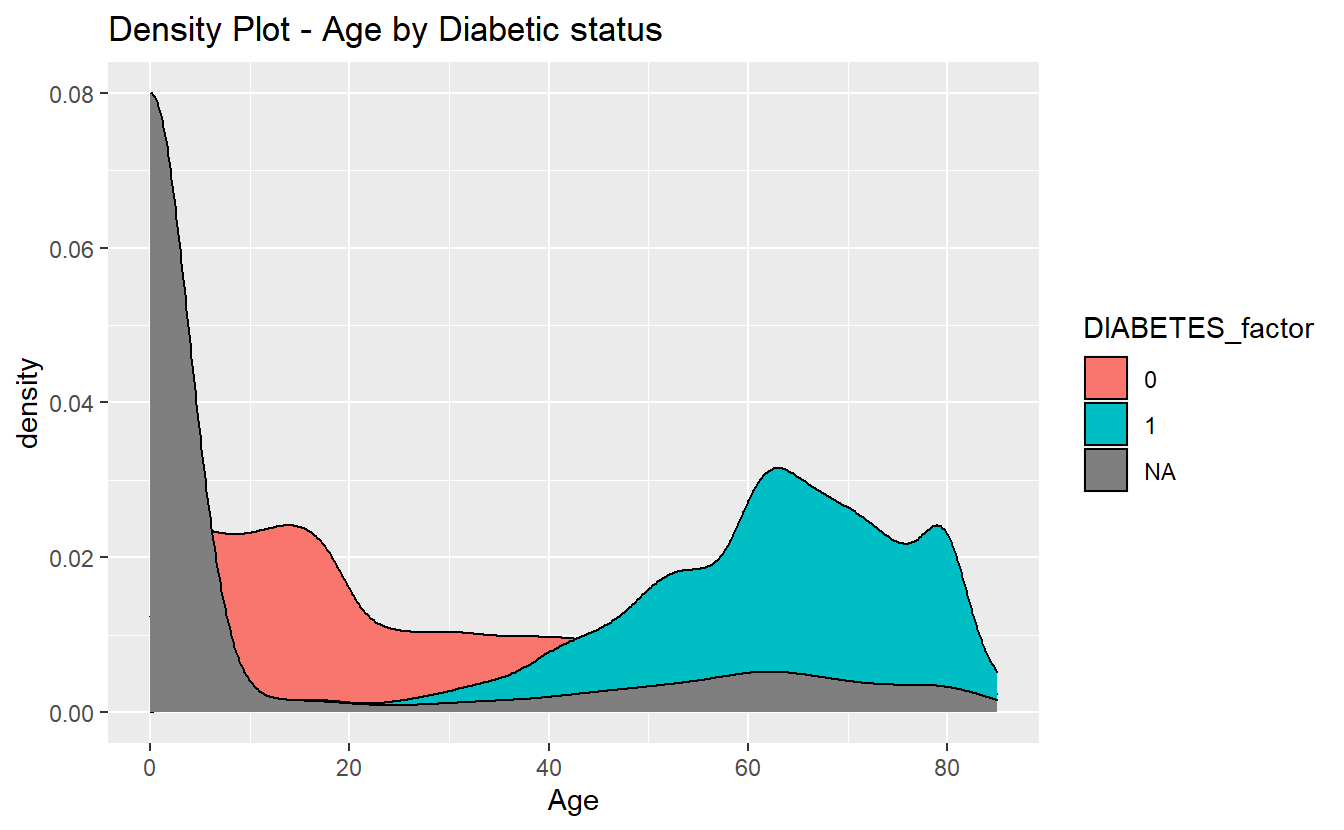plotly::ggplotly(density_plot_1) $$~$$ $$~$$ ## 6.5 Non-missing Data We can use Amelia::missmap to get a sense for how much of our data is missing: Amelia::missmap(as.data.frame(A_DATA))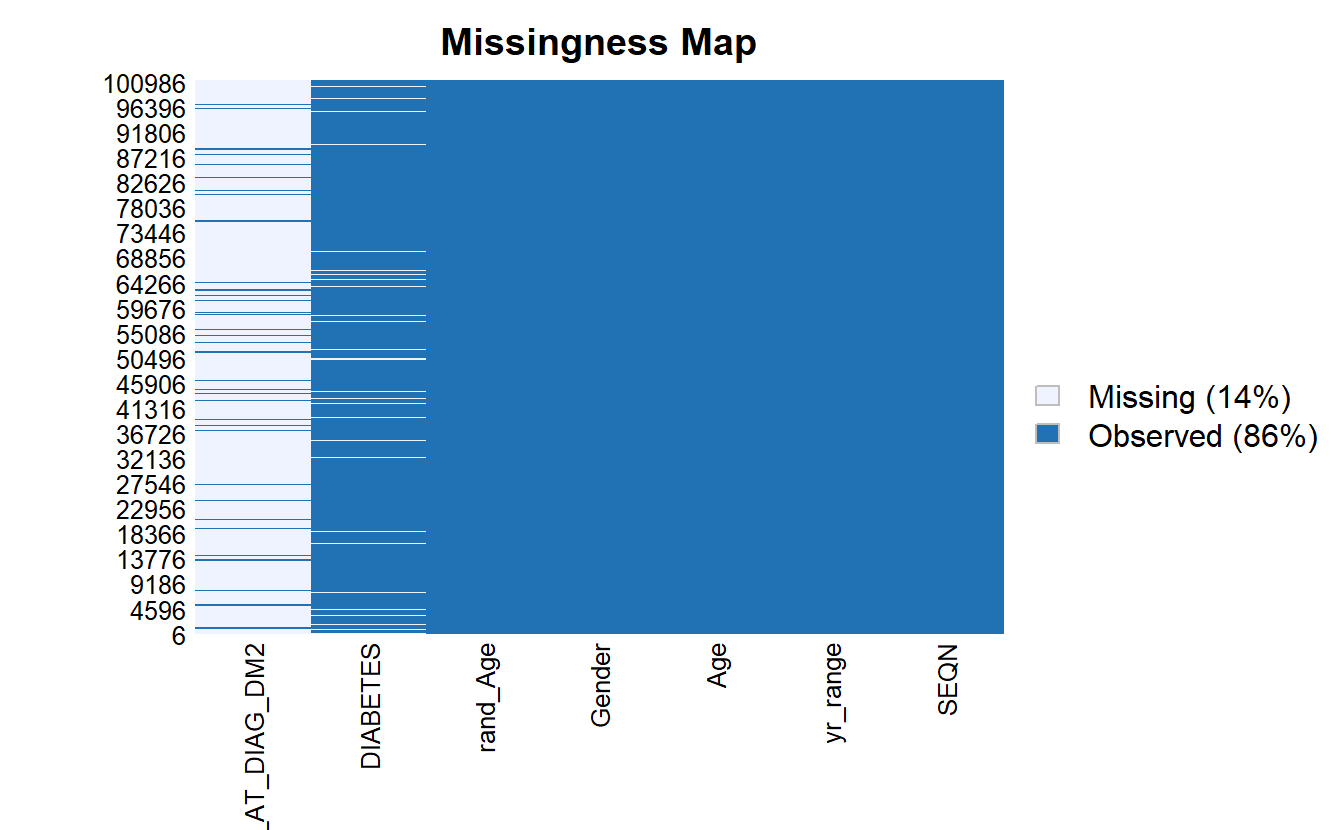For some analyses and models we need to remove missing data to prevent errors from R, for the reminder of this chapter, we will work with only the records that have non-missing values for SEQN, DIABETES, Age, and rand_Age: A_DATA.no_miss <- A_DATA %>% select(SEQN, DIABETES, Age, rand_Age) %>% mutate(DIABETES_factor = as.factor(DIABETES)) %>% mutate(DIABETES_factor = fct_relevel(DIABETES_factor, c('0','1') ) ) %>% na.omit() Notice now there are no missing values: Amelia::missmap(as.data.frame(A_DATA.no_miss))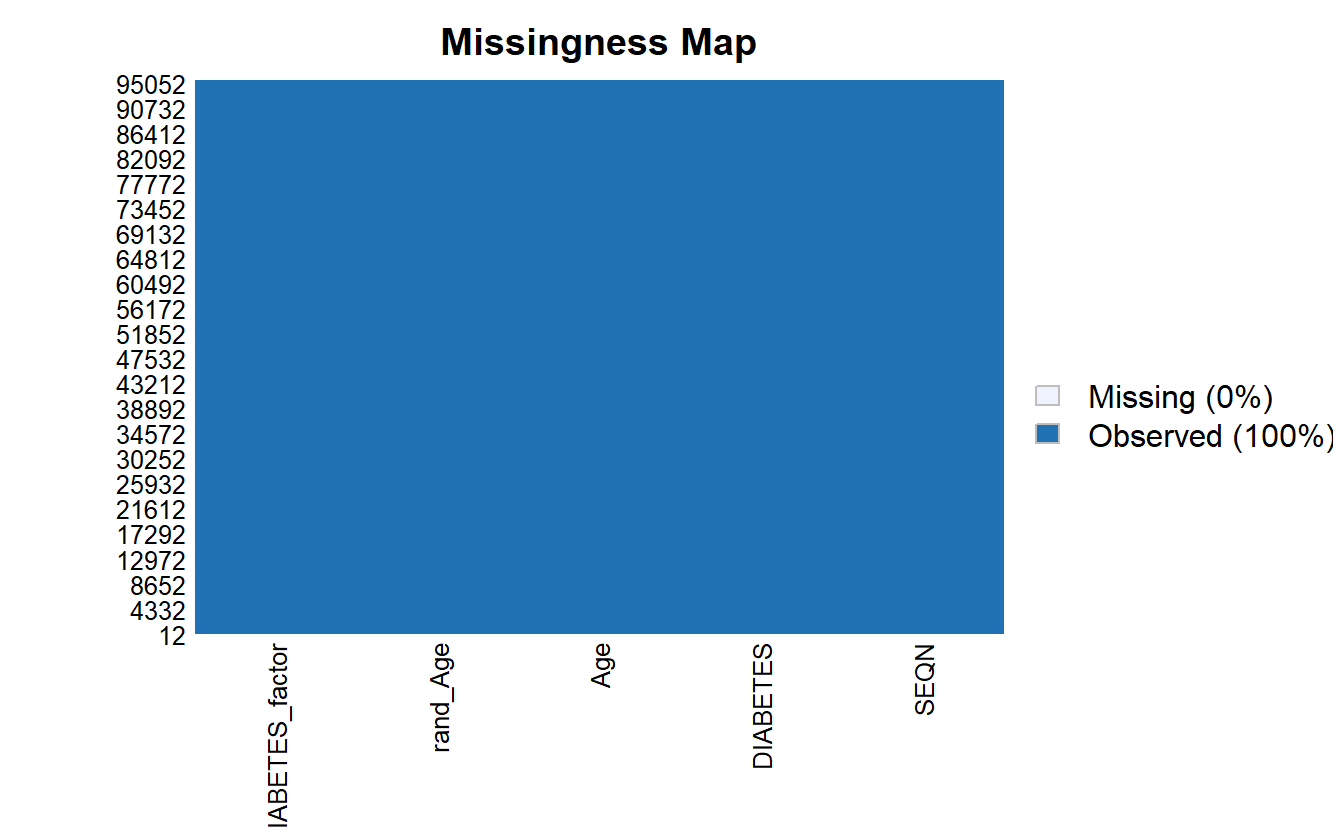$$~$$ $$~$$ ## 6.6 ANOVA Review Much like the t-test, Analysis of Variance (ANOVA) is used to determine whether there are any statistically significant differences between the means of two or more independent (unrelated) groups. ANOVA compares the means between two or more groups you that are interested in and determines whether any of the means of those groups are statistically significantly different from each other. Specifically, ANOVA tests the null hypothesis: $H_0 : \mu_1 = \mu_2 = \mu_2 = \cdots = \mu_f$ where $$\mu_i =$$ are the group means and $$f =$$ number of groups. If, however, the one-way ANOVA returns a statistically significant result ($$<.05$$ normally), we accept the alternative hypothesis, which is that there are at least two group means that are statistically significantly different from each other. res.aov <- aov(DIABETES ~ Age, data = A_DATA.no_miss) res.aov.sum <- summary(res.aov) res.aov.sum #> Df Sum Sq Mean Sq F value Pr(>F) #> Age 1 691 691.2 11729 <2e-16 *** #> Residuals 95545 5631 0.1 #> --- #> Signif. codes: 0 '***' 0.001 '**' 0.01 '*' 0.05 '.' 0.1 ' ' 1 Since this p-value is 0 $$<.05$$ we accept the alternative hypothesis, there is a statistically significantly difference between the two Age groups, just as we found when we performed the t-test. When we perform ANOVA for rand_Age on the data-set we find: aov(DIABETES ~ rand_Age, data = A_DATA.no_miss) %>% broom::tidy() #> # A tibble: 2 x 6 #> term df sumsq meansq statistic p.value #> <chr> <dbl> <dbl> <dbl> <dbl> <dbl> #> 1 rand_Age 1 0.000599 0.000599 0.00905 0.924 #> 2 Residuals 95545 6322. 0.0662 NA NA and, yes, broom::tidy also transformed our ANOVA output into a tibble this p-value for ANOVA with rand_Age is 0.9241969, NA $$>.05$$; therefore, we accept the null hypothesis that the two group means are approximately the same, and, again; this matches with what we found when we performed the t-test. $$~$$ $$~$$ ### 6.6.1 Split Data To test different models it is standard practice to split your data into a training set and a testing set, where: • the training set will be used to train the model and • the testing set or hold-out set will be used to evaluate model performance. While, we could also compute model evaluation metrics on the training set, the results will be overly-optimistic as the training set was used to train the model. In this example, we will sample 60% of the non-missing data’s member-ids without replacement: set.seed(123) sample.SEQN <- sample(A_DATA.no_miss$SEQN,
size = nrow(A_DATA.no_miss)*.6,
replace = FALSE)

# we can check that we got approximately 60% of the data:

length(sample.SEQN)/nrow(A_DATA.no_miss)
#>  0.5999979

Recall that the set.seed is there to ensure we get the same training and test set from run to run. We can always randomize if we use set.seed(NULL) or set.seed(Sys.time())

The training set will consist of those 57328 the 60% we chose at random:

A_DATA.train <- A_DATA.no_miss %>%
filter(SEQN %in% sample.SEQN)

And the testing set will consist of the remaining 40% of members not in sample.SEQN:

A_DATA.test <- A_DATA.no_miss %>%
filter(!(SEQN %in% sample.SEQN))

$$~$$

$$~$$

## 6.7 Logistic Regression

Let’s suppose our data-set contains a binary column $$y$$, where $$y$$ has two possible values: 1 and 0. Logistic Regression assumes a linear relationship between the features or predictor variables and the log-odds (also called logit) of a binary event.

This linear relationship can be written in the following mathematical form:

$\ell = \ln \left( \frac{p}{1-p} \right) = \beta_0 + \beta_1x_1 + \beta_2x_2 + \cdots + \beta_kx_k$

Where $$\ell$$ is the log-odds, $$\ln()$$ is the natural logarithm, and $$\beta_i$$ are coefficients for the features ($$x_i$$) of the model. We can solve the above equation for $$p$$:

$\frac{p}{1-p} = e^{\beta_0 + \beta_1x_1 + \beta_2x_2 + \cdots + \beta_kx_k}$

$p = (1-p)(e^{\beta_0 + \beta_1x_1 + \beta_2x_2 + \cdots + \beta_kx_k})$

$p = e^{\beta_0 + \beta_1x_1 + \beta_2x_2 + \cdots + \beta_kx_k} - p \cdot e^{\beta_0 + \beta_1x_1 + \beta_2x_2 + \cdots + \beta_kx_k}$

$p + p \cdot e^{\beta_0 + \beta_1x_1 + \beta_2x_2 + \cdots + \beta_kx_k} = e^{\beta_0 + \beta_1x_1 + \beta_2x_2 + \cdots + \beta_kx_k}$

$p(1 + e^{\beta_0 + \beta_1x_1 + \beta_2x_2 + \cdots + \beta_kx_k}) = e^{\beta_0 + \beta_1x_1 + \beta_2x_2 + \cdots + \beta_kx_k}$

$p = \frac{e^{\beta_0 + \beta_1x_1 + \beta_2x_2 + \cdots + \beta_kx_k}}{1 + e^{\beta_0 + \beta_1x_1 + \beta_2x_2 + \cdots + \beta_kx_k}}$

$p = \frac{1}{1+e^{-(\beta_0 + \beta_1x_1 + \beta_2x_2 + \cdots + \beta_kx_k)}}$

The above formula shows that once $$\beta_i$$ are known, we can easily compute either the log-odds that $$y=1$$ for a given observation, or the probability that $$y=1$$ for a given observation.

### 6.7.1 Odds Ratio

The odds of the dependent variable equaling a case ($$y=1$$), given some linear combination the features $$x_i$$ is equivalent to the exponential function of the linear regression expression.

$odds = e^{\beta_0 + \beta_1x_1 + \beta_2x_2 + \cdots + \beta_kx_k}$

This illustrates how the logit serves as a link function between the probability and the linear regression expression.

For a continuous independent variable the odds ratio can be defined as:

$OddsRatio = \frac{odds(x_j+1)}{odds(x_j)}$

Since we know what the odds are we can compute this as:

$OddsRatio = \frac{e^{\beta_0 + \beta_1x_1 + \cdots + \beta_j(x_j+1) + \cdots + \beta_kx_k}}{e^{\beta_0 + \beta_1x_1 + \cdots + \beta_j(x_j) + \cdots + \beta_kx_k}}$

$OddsRatio = \frac{e^{\beta_0}\cdot e^{\beta_1x_1}\cdots e^{\beta_j(x_j+1)}\cdots e^{\beta_kx_k}}{e^{\beta_0}\cdot e^{\beta_1x_1}\cdots e^{\beta_j(x_j)}\cdots e^{\beta_kx_k}}$

$OddsRatio = \frac{e^{\beta_j(x_j+1)}}{e^{\beta_jx_j}}$

$OddsRatio = e^{\beta_j}$

This exponential relationship provides an interpretation for the coefficents $$\beta_j$$:

For every 1-unit increase in $$x_j$$, the odds multiply by $$e^{\beta_j}$$.

### 6.7.2 Wald test

The Wald statistic is used to assess the significance of coefficients.

The Wald statistic is the ratio of the square of the regression coefficient to the square of the standard error of the coefficient; it is asymptotically distributed as a $$\chi^2$$ distribution:

$W_i = \frac{\beta_i}{SE_{\beta_i}^2}$

$$~$$

$$~$$

## 6.8 Train logistic regression with Age feature

In this example we will use the glm (General Linear Model) function, to train a logistic regression. A typical call to glm might include:

glm(formula,
data,
intercept = TRUE,
family = "#TODO" ,
...)

Where:

• formula - an object of class “formula” EX ( y ~ m*x + b , y ~ a + c + m*g + x^2)

• data - dataframe

• intercept - should an intercept be fit? TRUE/ FALSE

• … - glm has many other options see ?glm for others

• family - a description of the error distribution and link function to be used in the model. For glm this can be a character string naming a family function, a family function or the result of a call to a family function. Family objects provide a convenient way to specify the details of the models used by functions such as glm. Options include:

• binomial(link = "logit")

• the binomial family also accepts the links (as names): logit, probit, cauchit, (corresponding to logistic, normal and Cauchy CDFs respectively) log and cloglog (complementary log-log);
• gaussian(link = "identity")

• the gaussian family also accepts the links: identity, log , and inverse
• Gamma(link = "inverse")

• the Gamma family the links inverse, identity, and log
• inverse.gaussian(link = "1/mu^2")

• the inverse Gaussian family the links "1/mu^2", inverse, identity, and log.
• poisson(link = "log")

• the poisson family the links log, identity, and sqrt; and the inverse
• quasi(link = "identity", variance = "constant")

• quasibinomial(link = "logit")

• quasipoisson(link = "log")

• And the quasi family accepts the links logit, probit, cloglog, identity, inverse, log, 1/mu^2 and sqrt, and the function power can be used to create a power link function.

Below we fit our Logistic Regression with glm

toc <- Sys.time()

logit.DM2.Age <- glm(DIABETES ~ Age,
data = A_DATA.train,
family = "binomial")

tic <- Sys.time()

cat("Logistic Regression with 1 feature Age \n")
#> Logistic Regression with 1 feature Age
cat(paste0("Dataset has ", nrow(A_DATA.train), " rows \n"))
#> Dataset has 57328 rows
cat(paste0("trained in ", round(tic - toc, 4) , " seconds \n"))
#> trained in 0.7021 seconds

$$~$$

$$~$$

### 6.8.1 Logistic Regression Model Outputs

When we call the model we get a subset of output including:

1. the call to glm including the formula

2. Coefficients Table

• Estimate for coefficients ($$\beta_j$$)
3. Degrees of Freedom; Residual

4. Null Deviance

5. Residual Deviance / AIC

logit.DM2.Age
#>
#> Call:  glm(formula = DIABETES ~ Age, family = "binomial", data = A_DATA.train)
#>
#> Coefficients:
#> (Intercept)          Age
#>    -5.22504      0.05707
#>
#> Degrees of Freedom: 57327 Total (i.e. Null);  57326 Residual
#> Null Deviance:       29700
#> Residual Deviance: 23350     AIC: 23350

When we call summary on model object we often get some different out-put, in the case of glm:

summary(logit.DM2.Age)
#>
#> Call:
#> glm(formula = DIABETES ~ Age, family = "binomial", data = A_DATA.train)
#>
#> Deviance Residuals:
#>     Min       1Q   Median       3Q      Max
#> -1.0233  -0.3683  -0.1777  -0.1301   3.1990
#>
#> Coefficients:
#>               Estimate Std. Error z value Pr(>|z|)
#> (Intercept) -5.2250430  0.0534194  -97.81   <2e-16 ***
#> Age          0.0570724  0.0008558   66.69   <2e-16 ***
#> ---
#> Signif. codes:  0 '***' 0.001 '**' 0.01 '*' 0.05 '.' 0.1 ' ' 1
#>
#> (Dispersion parameter for binomial family taken to be 1)
#>
#>     Null deviance: 29698  on 57327  degrees of freedom
#> Residual deviance: 23350  on 57326  degrees of freedom
#> AIC: 23354
#>
#> Number of Fisher Scoring iterations: 6

We get the following

1. the call to glm including the formula

2. Deviance Residuals

3. Coefficients Table

• Estimate for coefficients ($$\beta_j$$)

• Standard Error

• z-value

• p-value

• Significance Level

4. Null Deviance

5. Residual Deviance

6. Akaike Information Criterion (AIC)

While this is all quite a lot of information, however, I find that the broom package does a much better job at collecting relevant information and parsing it out for review:

$$~$$

$$~$$

#### 6.8.1.1 Coefficients

As a default you could use

coef(logit.DM2.Age)
#> (Intercept)         Age
#> -5.22504305  0.05707239

In other words,

equatiomatic::extract_eq(logit.DM2.Age)

$\log\left[ \frac { P( \operatorname{DIABETES} = \operatorname{1} ) }{ 1 - P( \operatorname{DIABETES} = \operatorname{1} ) } \right] = \alpha + \beta_{1}(\operatorname{Age})$

equatiomatic::extract_eq(logit.DM2.Age, use_coefs = TRUE)

$\log\left[ \frac { \widehat{P( \operatorname{DIABETES} = \operatorname{1} )} }{ 1 - \widehat{P( \operatorname{DIABETES} = \operatorname{1} )} } \right] = -5.23 + 0.06(\operatorname{Age})$

however, if we use broom::tidy then the results will be a tibble, and we can easily add information such as the odds-ratio:

logit.DM2.Age.Coeff <- logit.DM2.Age %>%
broom::tidy() %>%
mutate(model = "Age") %>%
mutate(odds_ratio = if_else(term != '(Intercept)',
exp(estimate),
as.double(NA)))

We can use the kable function from the knitr package to print out a table for review

logit.DM2.Age.Coeff %>%
knitr::kable()
term estimate std.error statistic p.value model odds_ratio
(Intercept) -5.2250430 0.0534194 -97.81167 0 Age NA
Age 0.0570724 0.0008558 66.68565 0 Age 1.058732
library(knitr)

Going back to our interpretation of the Odds Ratio, for every 1 unit increase in Age our odds of getting Diabetes increases by about 5.8732448%

$$~$$

$$~$$

#### 6.8.1.2 Training Errors

Measures such as AIC, BIC, and Adjusted R2 are normally thought of training error estimates so you could group those into a table if you wanted. The broom::glance function will provide a table of training errors but if you want Adjusted R2 you will have to compute it yourself:

adj_r2 <- rsq::rsq(logit.DM2.Age)
#>  0.1024638

Then after glance converts the model training errors into a tibble we can use a mutate to add the additional column then we can print it for review:

logit.DM2.Age %>%
broom::glance() %>%
kable()
null.deviance df.null logLik AIC BIC deviance df.residual nobs adj_r2
29698.41 57327 -11674.89 23353.78 23371.69 23349.78 57326 57328 0.1024638

$$~$$

$$~$$

##### 6.8.1.2.1 Plots

These are default return plots for glm

###### 6.8.1.2.1.1 Residuals Vs Fitted
plot(logit.DM2.Age,1)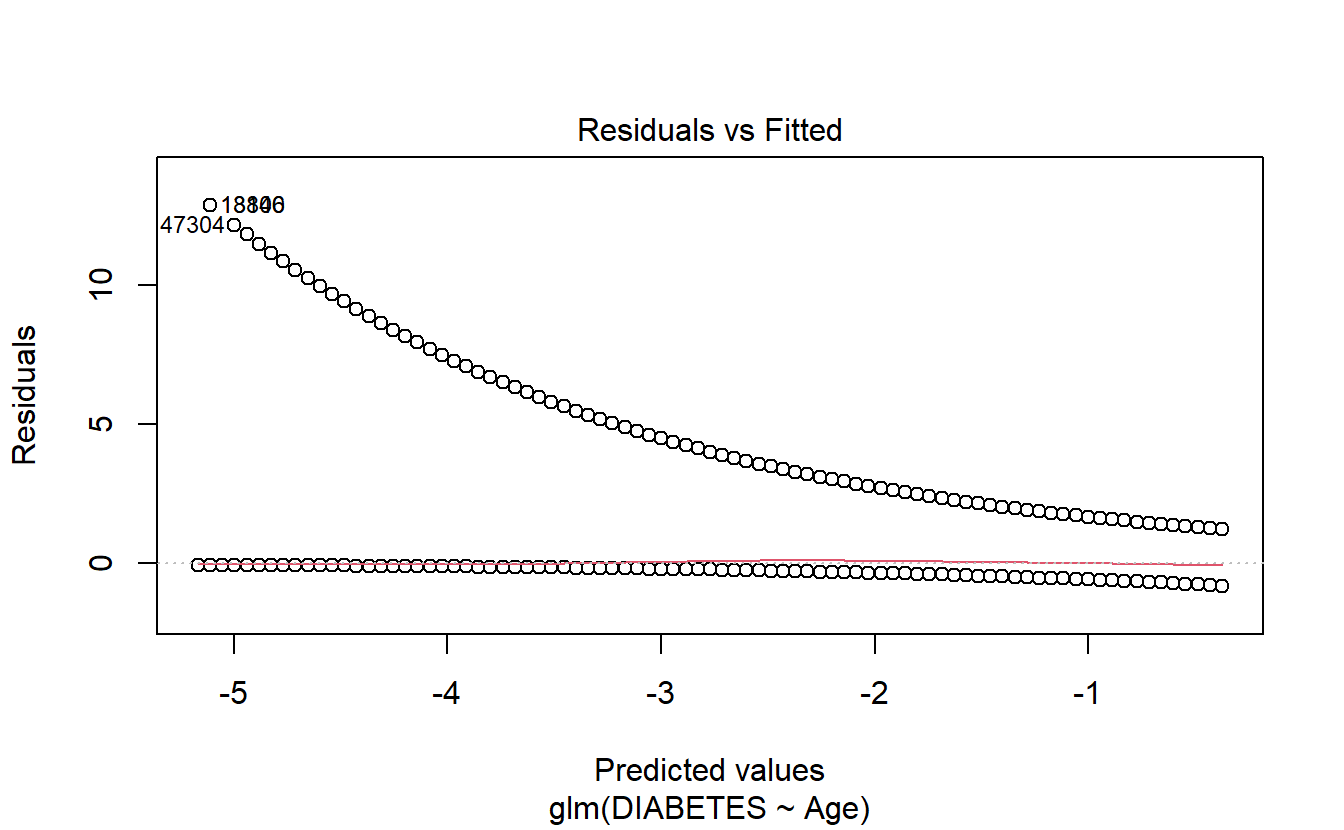###### 6.8.1.2.1.2 Q-Q Plot
plot(logit.DM2.Age,2)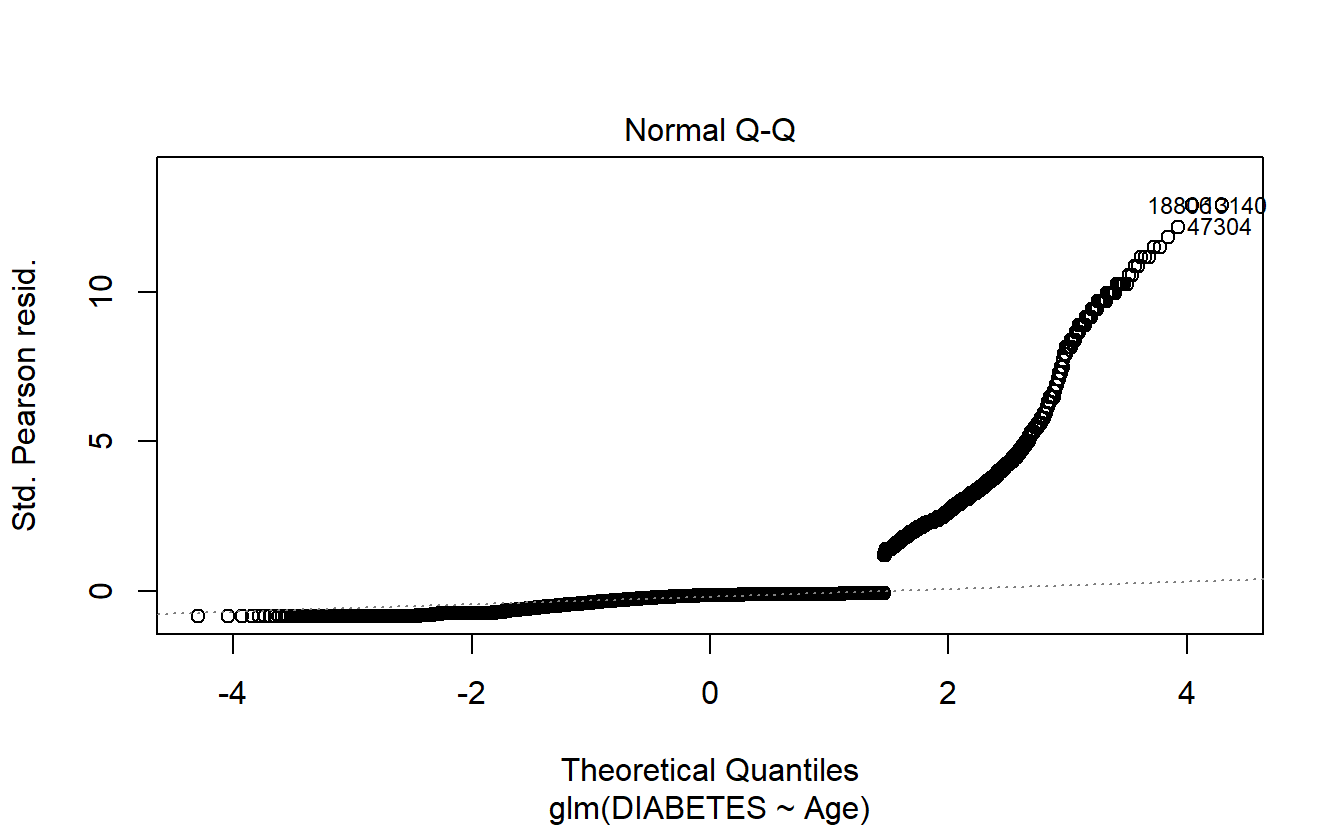###### 6.8.1.2.1.3 Scale-Location
plot(logit.DM2.Age,3)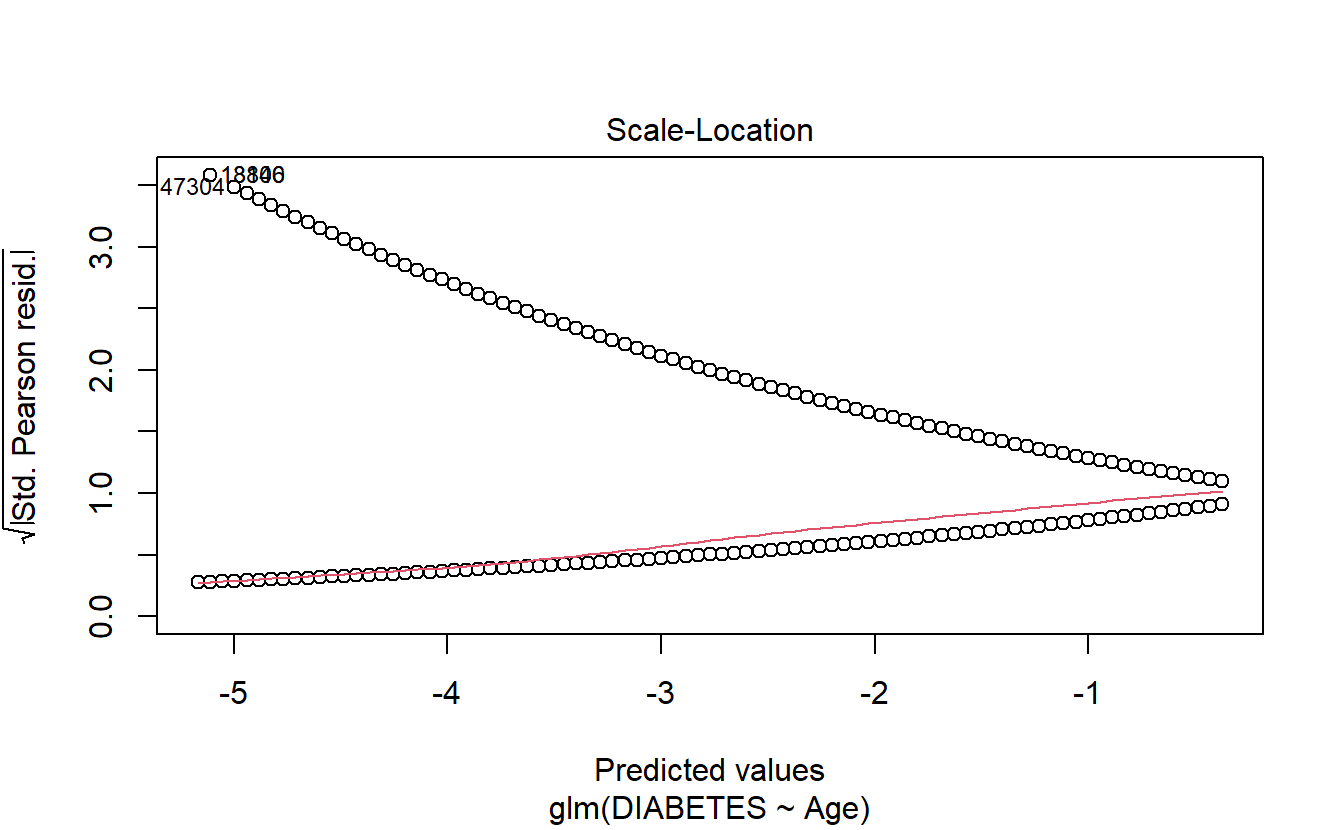###### 6.8.1.2.1.4 Cook’s distance
plot(logit.DM2.Age,4)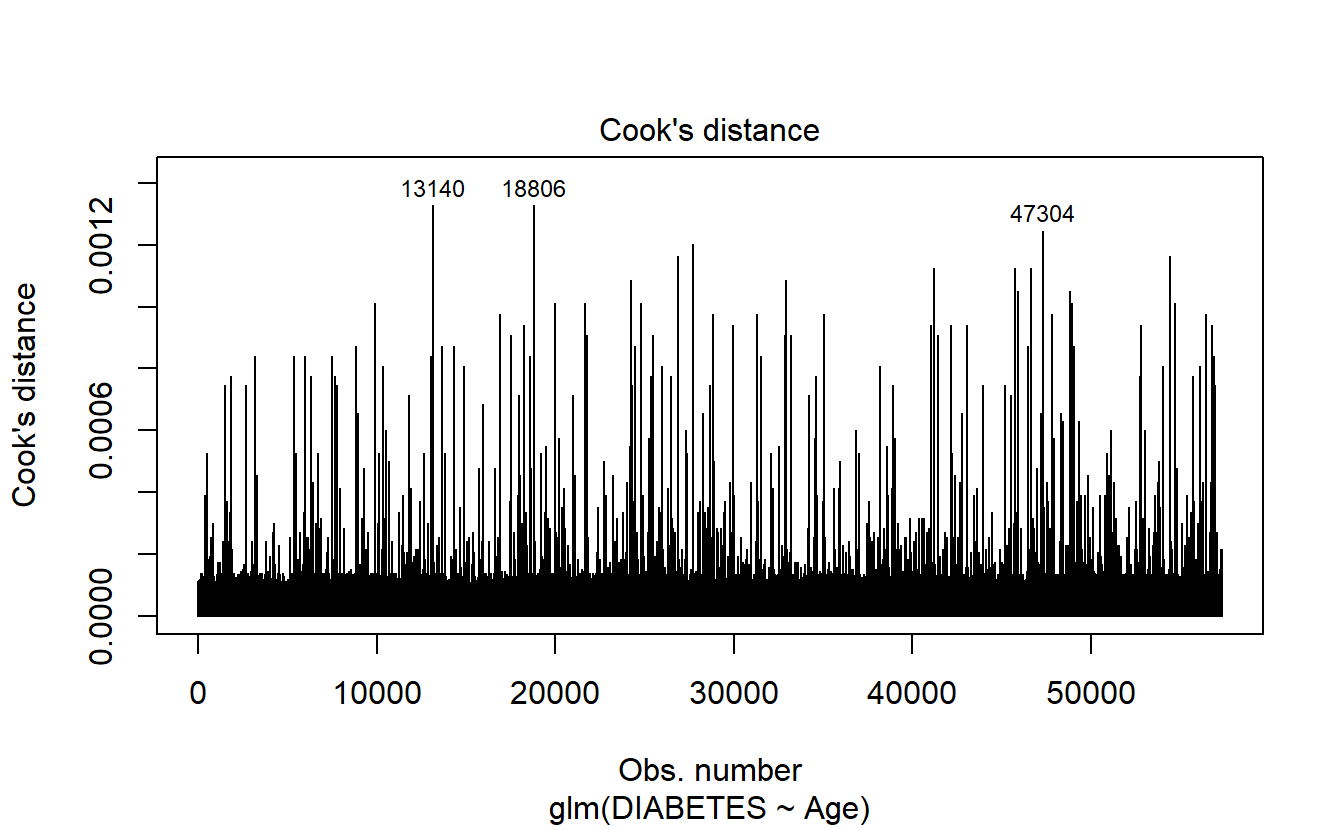###### 6.8.1.2.1.5 Leverage
plot(logit.DM2.Age,5)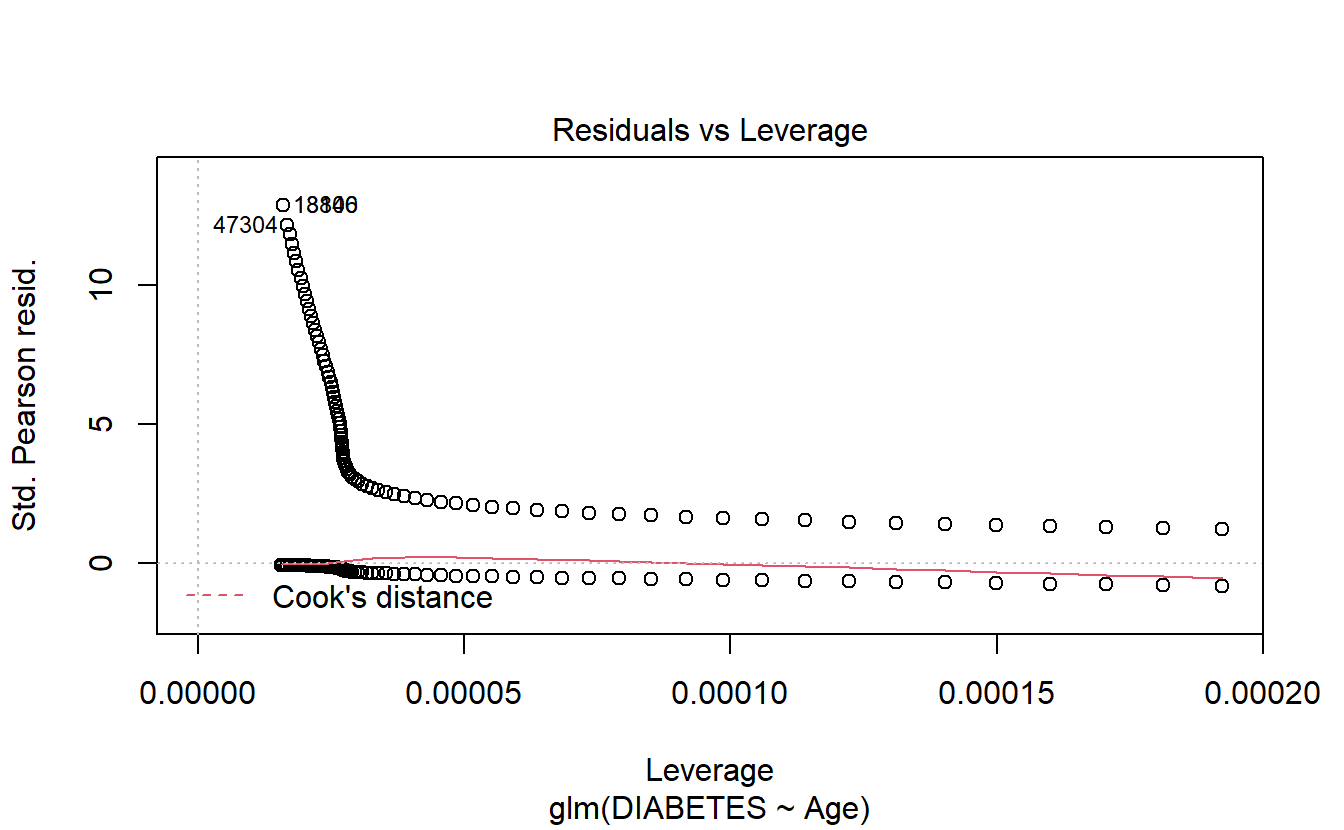###### 6.8.1.2.1.6 Cooks’s Vs Leverage
plot(logit.DM2.Age,6)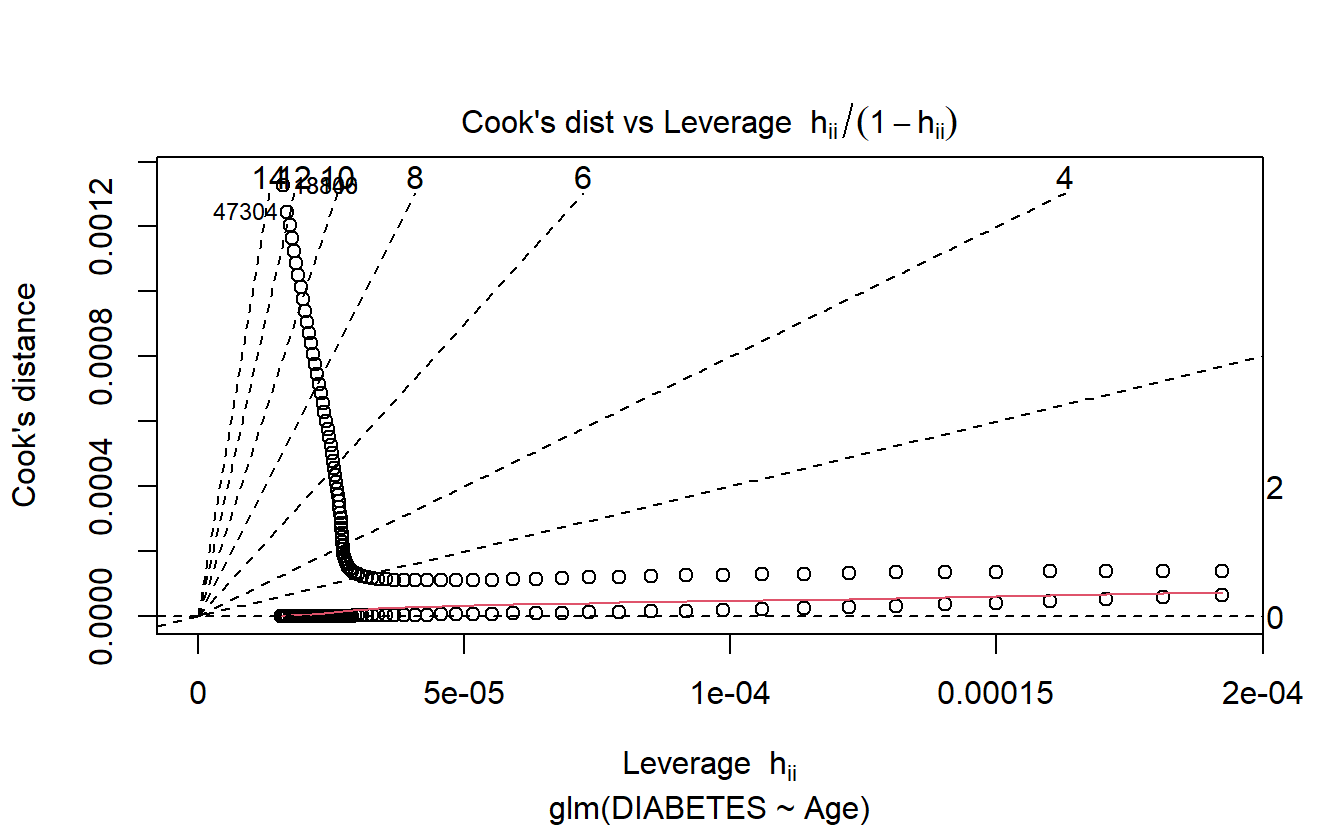$$~$$

$$~$$

## 6.9 Probability scoring Test Data with logit Age model

A_DATA.test.scored.DM2_Age <- A_DATA.test %>%
mutate(model = "logit_DM2_Age")

A_DATA.test.scored.DM2_Age$probs <- predict(logit.DM2.Age, A_DATA.test.scored.DM2_Age, "response") A_DATA.test.scored.DM2_Age %>% glimpse() #> Rows: 38,219 #> Columns: 7 #>$ SEQN            <dbl> 3, 4, 9, 11, 13, 14, 20, 25, 26, 27, 28, 29, 31, 39, 4~
#> $DIABETES <dbl> 0, 0, 0, 0, 1, 0, 0, 0, 0, 0, 0, 1, 0, 0, 0, 0, 0, 0, ~ #>$ Age             <dbl> 10, 1, 11, 15, 70, 81, 23, 42, 14, 18, 18, 62, 15, 7, ~
#> $rand_Age <dbl> 57, 60, 0, 57, 32, 23, 64, 31, 80, 65, 47, 72, 3, 2, 6~ #>$ DIABETES_factor <fct> 0, 0, 0, 0, 1, 0, 0, 0, 0, 0, 0, 1, 0, 0, 0, 0, 0, 0, ~
#> $model <chr> "logit_DM2_Age", "logit_DM2_Age", "logit_DM2_Age", "lo~ #>$ probs           <dbl> 0.009430610, 0.005663854, 0.009978965, 0.012506058, 0.~

$$~$$

$$~$$

## 6.10 Receiver Operating Characteristic Curve

The ROC curve is created by plotting the true positive rate (TPR) against the false positive rate (FPR) at various threshold settings.

The true-positive rate is also known as sensitivity, recall or probability of detection.

The false-positive rate is also known as probability of false alarm and can be calculated as $$(1 − specificity)$$.

library('yardstick')

NOTICE the following information that we get when we load the yardstick package:

For binary classification, the first factor level is assumed to be the event. Use the argument event_level = "second" to alter this as needed.

Recall that our levels for DIABETES_factor are:

levels(A_DATA.no_miss$DIABETES_factor) #>  "0" "1" In other words, currently our event-level is the second option, 1! Note that the return of roc_curve is a tibble containing: .threshold , specificity, sensitivity: A_DATA.test.scored.DM2_Age %>% roc_curve(truth= DIABETES_factor, probs, event_level = "second") #> # A tibble: 87 x 3 #> .threshold specificity sensitivity #> <dbl> <dbl> <dbl> #> 1 -Inf 0 1 #> 2 0.00566 0 1 #> 3 0.00599 0.0338 1.00 #> 4 0.00634 0.0675 0.999 #> 5 0.00671 0.0896 0.999 #> 6 0.00711 0.114 0.999 #> # ... with 81 more rows The Area Under the ROC Curve or c-statistic or AUC is the area under the the ROC curve can also be computed. AUC.DM2_Age <- A_DATA.test.scored.DM2_Age %>% roc_auc(truth = DIABETES_factor, probs, event_level = "second") AUC.DM2_Age #> # A tibble: 1 x 3 #> .metric .estimator .estimate #> <chr> <chr> <dbl> #> 1 roc_auc binary 0.843 in this case our AUC is 0.8427198. Notice that the return to roc_auc is a tibble with columns: .metric .estimator and .estimate. We could graph the ROC curve using ggplot2: A_DATA.test.scored.DM2_Age %>% roc_curve(truth = DIABETES_factor, probs, event_level = "second") %>% mutate(FPR = 1-specificity) %>% mutate(TPR = sensitivity) %>% ggplot(aes(x=FPR, y=TPR, color=.threshold)) + geom_point() + scale_colour_gradientn(colours=rainbow(4)) + geom_abline(slope = 1) + labs(title = "ROC Curve " , subtitle = 'test set scored on logistic regression with Age feature', caption = paste0("AUC : ", round(AUC.DM2_Age$.estimate,3)))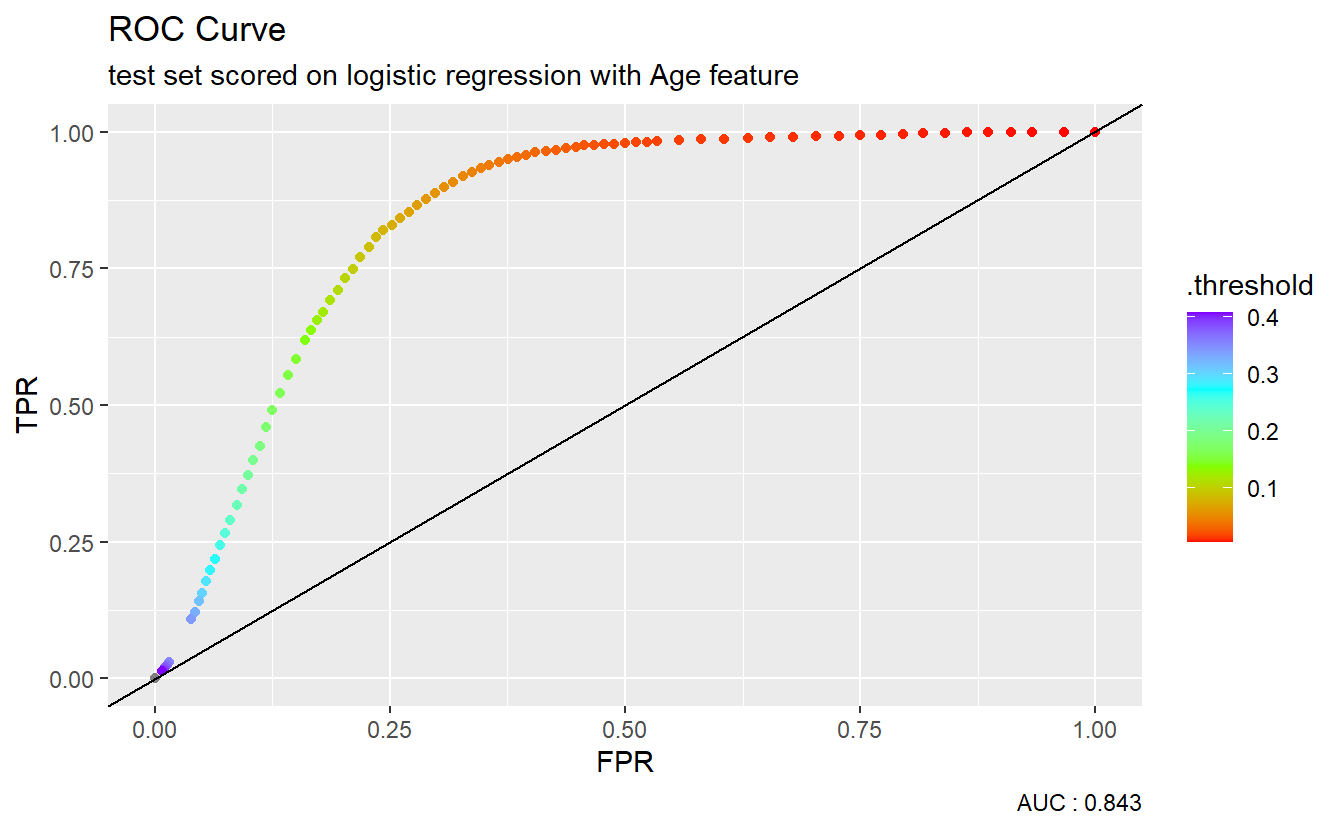However, many metrics within the yardstick package can call to a pre-specified autoplot function so a call to an ROC curve can be as easy as:

A_DATA.test.scored.DM2_Age %>%
roc_curve(truth = DIABETES_factor, probs, event_level = "second") %>%
autoplot() +
labs(title = "ROC Curve " ,
subtitle = 'test set scored on logistic regression with Age feature',
caption = paste0("AUC : ", round(AUC.DM2_Age$.estimate,3)))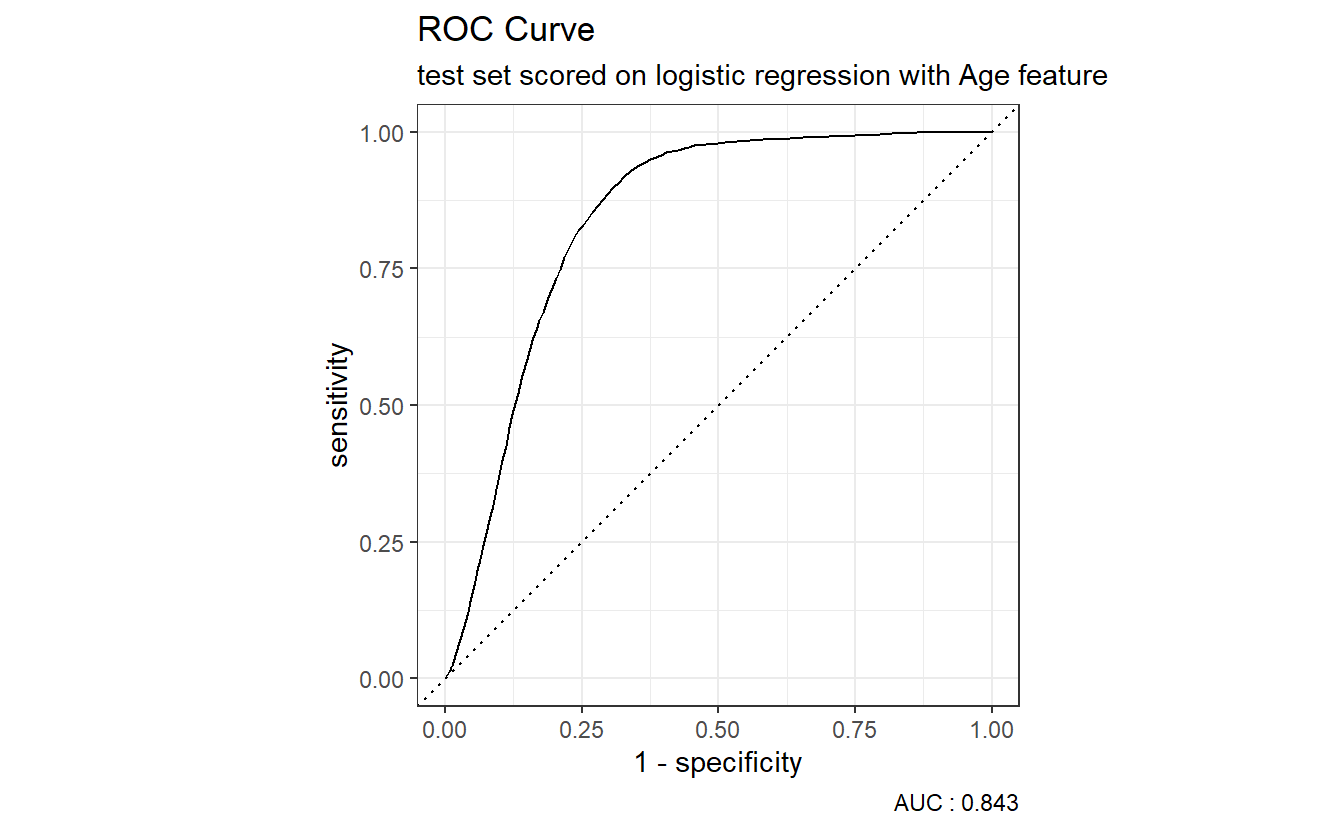The dotted diagonal line above represents the ROC curve of a “Coin-flip” model, in general the higher AUCs are indicative of better performing models. $$~$$ $$~$$ ## 6.11 Setting a Threshold We will set our threshold to be the mean probability of Diabetes population in the training set: A_DATA.train.scored <- A_DATA.train A_DATA.train.scored$probs <- predict(logit.DM2.Age,
A_DATA.train,
"response")
A_DATA.train.scored %>%
ggplot(aes(x=Age,y=probs, color=DIABETES_factor)) +
geom_point() +
geom_line()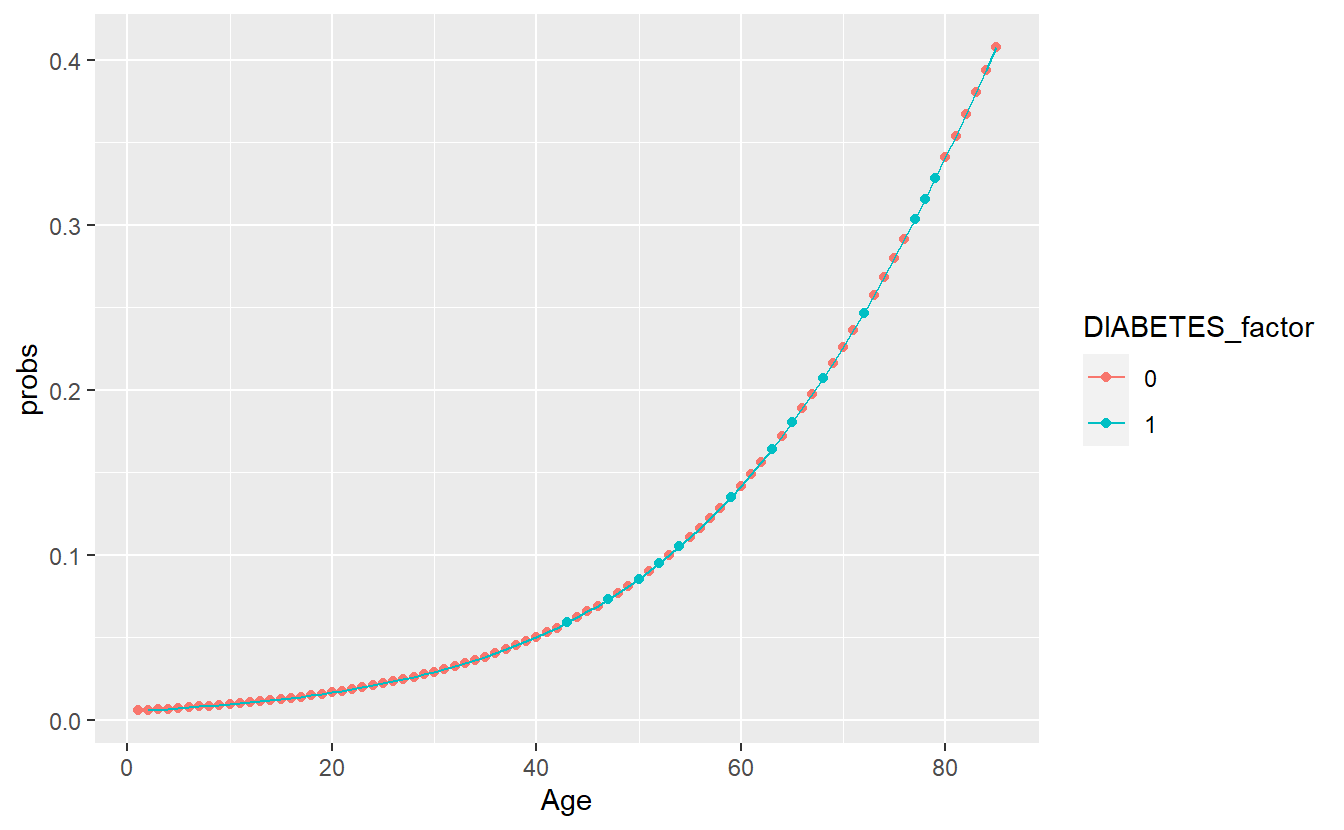DM2_Age.prob_sum <- A_DATA.train.scored %>%
group_by(DIABETES_factor) %>%
summarise(min_prob = min(probs),
mean_prob = mean(probs),
max_prob = max(probs))

threshold_value <- (DM2_Age.prob_sum %>% filter(DIABETES_factor == 1))$mean_prob DM2_Age.prob_sum #> # A tibble: 2 x 4 #> DIABETES_factor min_prob mean_prob max_prob #> <fct> <dbl> <dbl> <dbl> #> 1 0 0.00566 0.0636 0.408 #> 2 1 0.00599 0.182 0.408 In the scoring set, if the probability is greater than our threshold of 0.1815463 then we will predict a label of 1 otherwise 0: A_DATA.test.scored.DM2_Age <- A_DATA.test.scored.DM2_Age %>% mutate(pred = if_else(probs > threshold_value, 1, 0)) %>% mutate(pred_factor = as.factor(pred)) %>% mutate(pred_factor = fct_relevel(pred_factor, c('0','1') )) %>% mutate(correct_guess = if_else(pred_factor == DIABETES_factor,TRUE,FALSE)) A_DATA.test.scored.DM2_Age %>% ggplot(aes(x=Age , y=probs, color=correct_guess)) + geom_point() + geom_hline(yintercept = threshold_value) + facet_wrap(DIABETES_factor ~ .)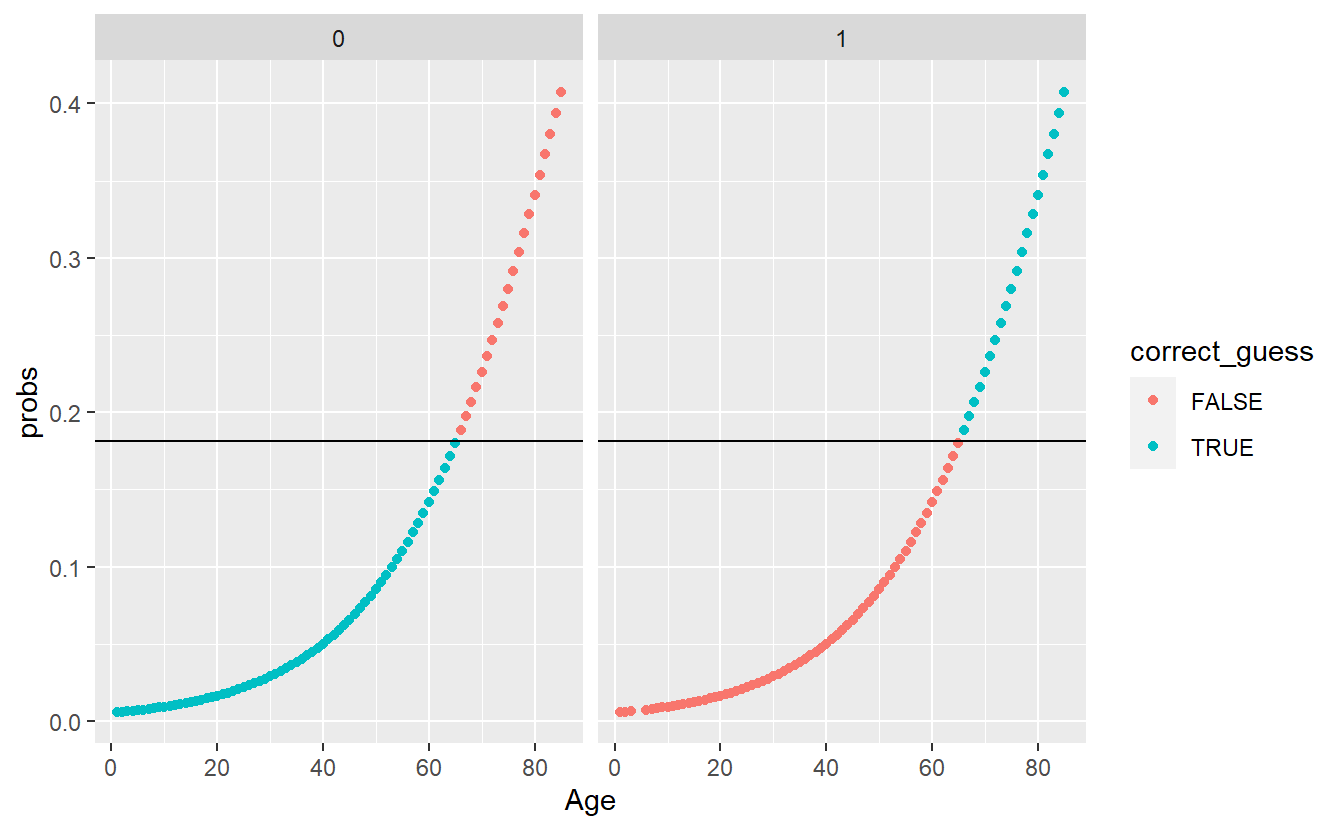To further evaluate the above we will make use of a confusion matrix. $$~$$ $$~$$ ## 6.12 Confusion Matrix A confusion matrix is a table consisting of counts of predicted versus actual classes: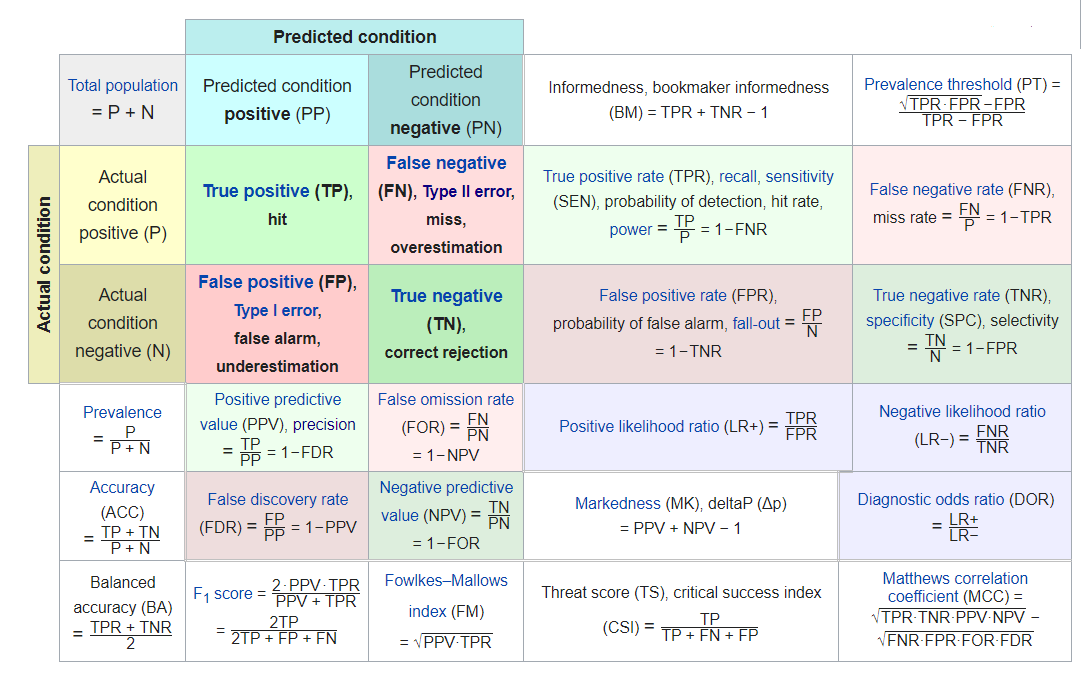conf_mat.Age <- A_DATA.test.scored.DM2_Age %>% conf_mat(truth = DIABETES_factor, pred_factor) conf_mat.Age #> Truth #> Prediction 0 1 #> 0 31569 1535 #> 1 3976 1139 Above we see that we have: TP <- conf_mat.Age$table[2,2]
TN <- conf_mat.Age$table[1,1] FP <- conf_mat.Age$table[2,1]
FN <- conf_mat.Age\$table[1,2]
• True Positives : 1139
• True Negatives : 31569
• False Positive / False Alarm: 3976
• False Negative : 1535

so we could fill in this confusion matrix:

Confusion Matrix
True Condition
Total Population Condition Positive Condition Negative Prevalence

Accuracy

accuracy <- (TP + TN)/(TP + TN + FP + FN)

Balanced Accuracy

bal_accuracy <- (TPR + TNR)/2

Predicted Predicted Positive

True Positive

TP

False Positive

FP

PPV, Precision

PPV <- TP/(TP+FP)

FDR
Condition Predicted Negative

False Negative

FN

True Negative

TN

FOR NPV

TPR, Recall

TPR <- TP/(TP+FN)

FPR LR_P DOR MCC
FNR

TNR

TNR <- TN/(TN+FP)

LR_N

F1

f1 <- 2*((Precision * Recall)/(Precision + Recall))

There are a few autoplots associated with the confusion matrix worth noting. A heatmap plot:

conf_mat.Age %>%
autoplot('heatmap')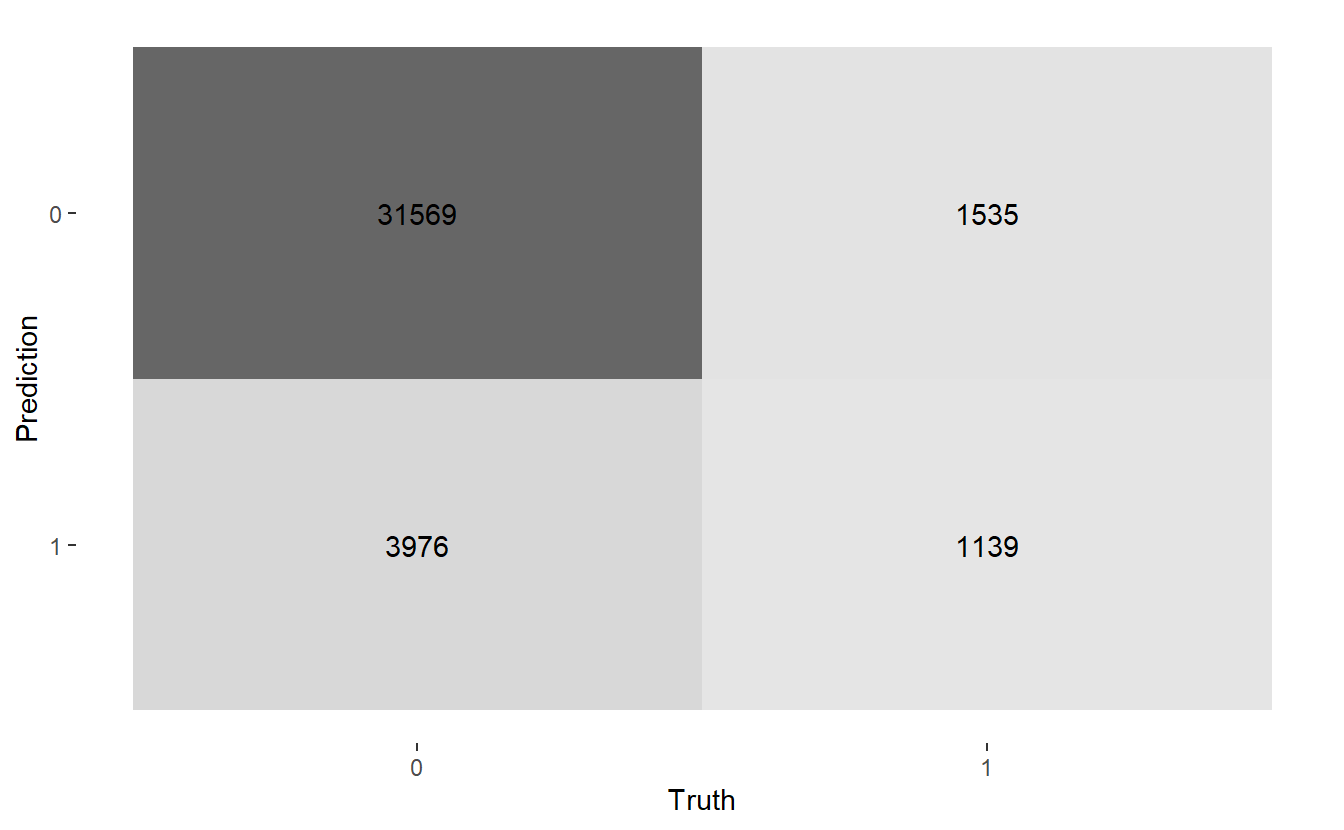And a mosaic plot:

conf_mat.Age %>%
autoplot('mosaic')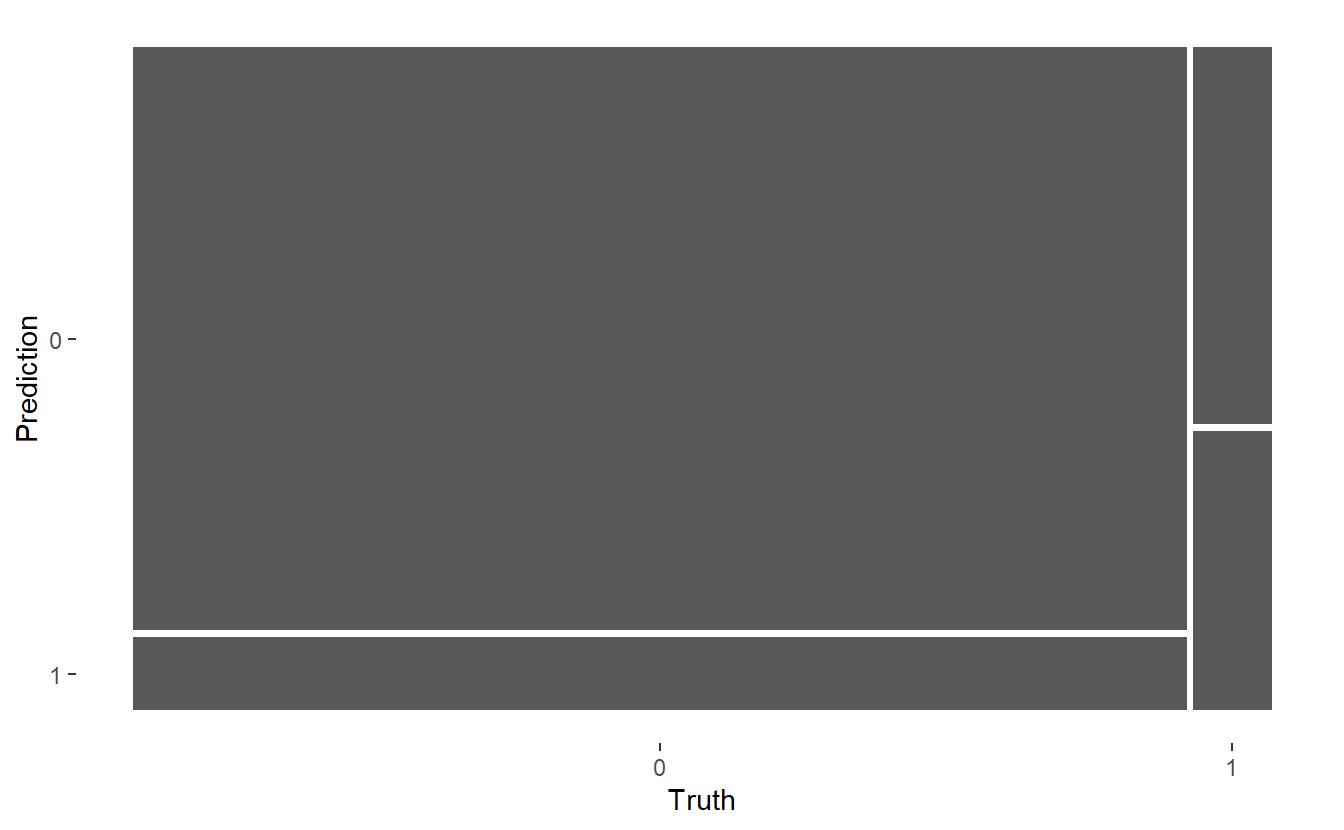$$~$$

$$~$$

## 6.13 Model Evaluation Metrics

From a review of the various Model Evaluation Metrics formula we can code corresponding model metrics of interest for this confusion matrix:

accuracy <- (TP + TN)/(TP + TN + FP + FN)

Prevalence <- (TP + FN)/(TP + TN + FP + FN)

#TPR = Recall, Sensitivity
TPR <- TP/(TP+FN)

#Specificity
TNR <- TN/(TN+FP)

Precision <- TP/(TP+FP)
Recall <- TP/(TP+FN)

bal_accuracy <- (TPR + TNR)/2

f1 <- 2*((Precision*Recall)/(Precision+Recall))

tibble(TPR, Recall, TNR, Precision, accuracy , Prevalence ,  bal_accuracy, f1)
#> # A tibble: 1 x 8
#>     TPR Recall   TNR Precision accuracy Prevalence bal_accuracy    f1